JP5612826B2 - Game program and game device - Google Patents

Game program and game device Download PDF

Info

Publication number
JP5612826B2
JP5612826B2 JP2009096501A JP2009096501A JP5612826B2 JP 5612826 B2 JP5612826 B2 JP 5612826B2 JP 2009096501 A JP2009096501 A JP 2009096501A JP 2009096501 A JP2009096501 A JP 2009096501A JP 5612826 B2 JP5612826 B2 JP 5612826B2
Authority
JP
Japan
Prior art keywords
posture
reference plane
input device
plane
based
Prior art date
Legal status (The legal status is an assumption and is not a legal conclusion. Google has not performed a legal analysis and makes no representation as to the accuracy of the status listed.)
Active
Application number
JP2009096501A
Other languages
Japanese (ja)
Other versions
JP2010246610A (en
Inventor

Original Assignee

Priority date (The priority date is an assumption and is not a legal conclusion. Google has not performed a legal analysis and makes no representation as to the accuracy of the date listed.)
Filing date
Publication date
Application filed by 任天堂株式会社 filed Critical 任天堂株式会社
Priority to JP2009096501A priority Critical patent/JP5612826B2/en
Priority claimed from US12/539,957 external-priority patent/US9427657B2/en
Publication of JP2010246610A publication Critical patent/JP2010246610A/en
Application granted granted Critical
Publication of JP5612826B2 publication Critical patent/JP5612826B2/en
Application status is Active legal-status Critical
Anticipated expiration legal-status Critical

Images

•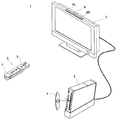•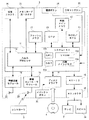•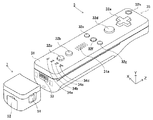•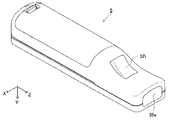•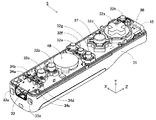•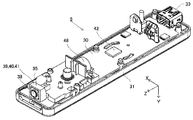•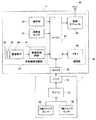•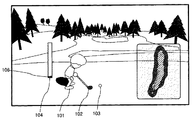•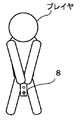•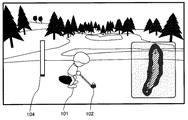•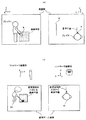•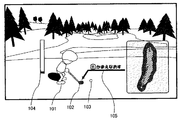••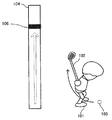•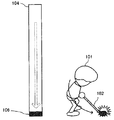•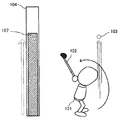•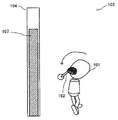••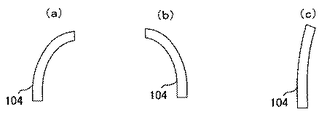•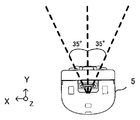•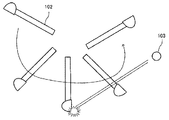•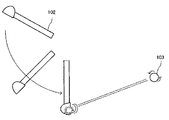•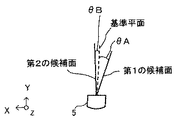•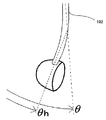•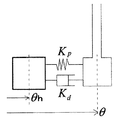•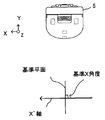•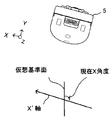•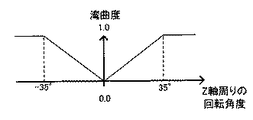•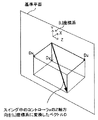•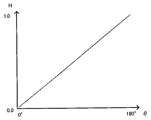•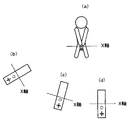•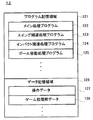•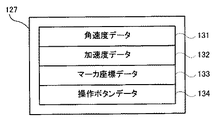•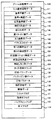•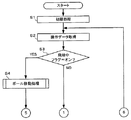•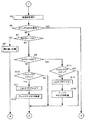•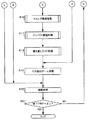•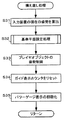•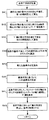•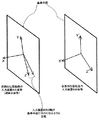••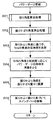•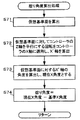•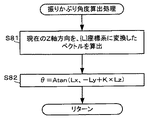•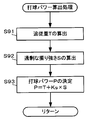•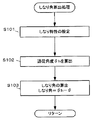•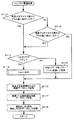•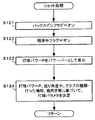•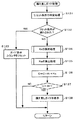•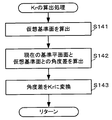•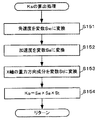•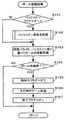•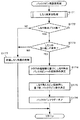••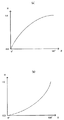•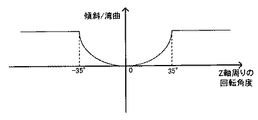•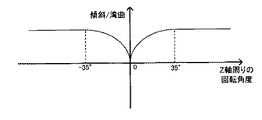•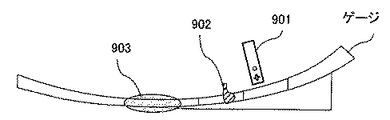Description

The present invention relates to a game program for executing a game process based on posture movement information acquired from predetermined detection means for detecting the posture or movement of an input device operated by a user, and more specifically, to a game device. The present invention relates to a game program and a game apparatus for moving an object in a virtual space based on posture movement information.

Conventionally, in a so-called golf game, a golf game that is enjoyed by operating a golf club in a virtual game space (golf swing) by performing an operation of swinging up and down the wireless controller itself (for example, golf swing) is disclosed (for example, Non-patent document 1). In the golf game, the flow of operations when swinging is as follows. First, a gauge as shown in FIG. 58 is displayed on the screen. On the gauge, a remote controller cursor 901, a club head cursor 902, and an impact zone 903 are displayed. Then, the player swings up the controller while pressing the A button of the controller. Then, in conjunction with this movement, the remote control cursor 901 in the screen moves along the gauge (moves in the right direction in FIG. 58). At the same time, the club head cursor 902 moves so as to follow the remote control cursor 901. Thereafter, when the club head cursor 902 catches up and overlaps the remote control cursor 901, the club head cursor 902 is reversed and returns to the original position. When the club head cursor 902 returns to within the range of the impact zone 903, the player can swing the controller to perform a golf shot operation.

"WE LOVE GOLF!" Instruction Manual, CAPCOM, P6-7, P16-17, P22-23

However, the game apparatus as described above has the following problems. In the golf game described above, it is assumed that the controller is held and the direction in which it is raised is in accordance with predetermined contents. (In other words, the way the controller is held and the direction in which it is raised is a fixed content.) Then, on the premise that the movement according to the predetermined content is performed, the shot determination in the impact zone 903 is performed. Processing is being executed. However, there are individual differences depending on the player in terms of how to hold the controller and the direction in which it is raised. Therefore, depending on the player, even if the player intends to perform the same action, the action may not be in accordance with the above-described predetermined contents. As a result, the shot may not be recognized, or conversely There was a problem that erroneous recognition of shots occurred.

SUMMARY OF THE INVENTION Therefore, an object of the present invention is to provide a game program and a game apparatus that can further reduce misjudgment and misrecognition in the determination of golf game shots.

The present invention employs the following configuration in order to solve the above problems. Note that the reference numerals in parentheses, supplementary explanations, and the like are examples of the correspondence with the embodiments described later in order to help understanding of the present invention, and do not limit the present invention.

The first invention is a game program that is executed by a computer of a game device that moves an object in a virtual space based on posture movement information acquired from predetermined detection means for detecting the posture or movement of an input device operated by a user. The computer is caused to function as posture motion information acquisition means (S2), posture calculation means (S31), reference plane setting means (S32), and processing means (S17). The posture movement information acquisition unit acquires the posture movement information detected by the detection unit. The posture calculation means calculates the posture of the input device based on the posture motion information. The reference plane setting means virtually sets the predetermined plane as a reference plane in the real space based on the posture movement information at a predetermined timing. The processing means executes a predetermined process corresponding to the attitude of the input device with respect to the reference plane.

According to the first aspect, it is possible to reduce misrecognition and misjudgment of the player's operation.

In a second aspect based on the first aspect, the predetermined detecting means is a predetermined motion sensor provided in the input device.

According to the second invention, the attitude of the input device can be calculated more accurately.

In a third aspect based on the first aspect, the reference plane setting means sets the reference plane based on the attitude of the input device calculated by the attitude calculation means.

According to the third aspect, since the reference plane is set based on the attitude of the input device held by the player, it is possible to further reduce misrecognition and misjudgment of the player's operation.

In a fourth aspect based on the third aspect, the reference plane setting means sets the reference plane based on the posture when the user operates the input device.

According to the fourth aspect of the invention, it is possible to cause the user to perform a reference plane setting operation at an arbitrary timing.

In a fifth aspect based on the third aspect, the game program causes the computer to further function as angle calculation means and determination means. The angle calculation means calculates an angle formed between the attitude of the input device and the reference plane. The determination unit determines whether or not an angle formed between the attitude of the input device and the reference plane satisfies a predetermined condition. The processing means executes a predetermined process when the determination means determines that the predetermined condition is satisfied.

In a sixth aspect based on the fifth aspect, the determining means determines whether the attitude of the input device and the reference plane are horizontal based on the angle calculated by the angle calculating means.

According to a seventh aspect based on the fifth aspect, the game program, until the determination means determines that the angle formed between the posture of the input device and the reference plane satisfies a predetermined condition, The processing by the posture calculation unit, the angle calculation unit, and the determination unit is repeatedly executed.

In an eighth aspect based on the seventh aspect, the determining means determines whether or not the sign of the angle formed by the attitude of the input device and the reference plane is inverted based on the angle calculated by the angle calculating means. .

According to the fifth to eighth inventions, for example, when the player performs an action such as a golf swing, the operation intended by the player can be more accurately reflected in the game process.

In a ninth aspect based on the eighth aspect, the input device defines three orthogonal axes. Then, the reference plane setting unit sets the reference plane based on the postures in the predetermined two-axis directions among the three defined axes in the posture of the input device calculated by the posture calculation unit.

According to a tenth aspect, in the ninth aspect, the reference plane setting means calculates candidate plane calculation means (S41, S43) for calculating two planes for setting the reference plane based on respective postures in two predetermined biaxial directions. )including. The reference plane setting means sets the reference plane based on the two planes calculated by the candidate plane calculation means.

According to the ninth to tenth aspects, the reference plane can be calculated from various viewpoints.

In an eleventh aspect based on the tenth aspect, the candidate plane calculating means calculates a plane including a predetermined one of the three defined axes and the direction of gravity as one of the two planes. .

In a twelfth aspect based on the eleventh aspect, the candidate plane calculating means calculates a plane including the axis defined as the longitudinal axis of the input device and the gravity direction as one of the two planes. .

In a thirteenth aspect based on the tenth aspect, the candidate plane calculating means calculates a plane including the axis defined as the vertical axis of the input device and the direction of gravity as one of the two planes. .

In a fourteenth aspect based on the tenth aspect, the candidate plane calculating means is defined as a vertical axis of the input device that rotates the axis defined as the longitudinal axis of the input device in the direction of gravity. A plane including the virtual axis and the direction of gravity calculated by adding to the axis is calculated as one of the two planes.

In a fifteenth aspect based on the tenth aspect, the reference plane setting means weights the two planes calculated by the candidate plane calculation means based on predetermined conditions, and calculates a weighted average of the two planes. Is calculated and set as a reference plane.

In a sixteenth aspect based on the fifteenth aspect, the reference plane setting means is heavier than the plane that is closer to the reference plane set immediately before, among the two planes calculated by the candidate plane calculation means. Perform weighting.

According to a seventeenth aspect, in the fifteenth aspect, the reference plane setting unit is configured to calculate a plane calculated based on an axis that is farther from the direction of gravity among the two planes calculated by the candidate plane calculation unit. Weigh more heavily.

According to the eleventh to seventeenth aspects, the reference plane can be calculated from various viewpoints using two planes, and the calculation for calculating the reference plane is unstable due to the difference in how the controller is held. Can be avoided.

In an eighteenth aspect based on the ninth aspect, the reference plane setting means selects, as a calculation axis, an axis farther from the gravitational direction among predetermined two axial directions defined in the input device. Then, the reference plane is set based on the posture applied to the calculation axis.

In a nineteenth aspect based on the eighteenth aspect, the reference plane setting means sets the calculation axis plane based on the calculation axis. The reference plane setting means sets the reference plane based on the calculation axial plane.

In a twentieth aspect based on the nineteenth aspect, the reference plane setting means sets the reference plane based on the old reference plane which is the reference plane set immediately before and the calculation axis plane.

In a twenty-first aspect based on the twentieth aspect, the reference plane setting means weights each of the old reference plane and the calculation axis plane based on a predetermined condition, and calculates a weighted average of the two planes. Is calculated and set as a reference plane.

In a twenty-second aspect based on the twenty-first aspect, the reference plane setting means weights the old reference plane more heavily.

In a twenty-third aspect based on the twenty-first aspect, the reference plane setting means weights more heavily as the calculation axis is located farther from the direction of gravity.

According to the eighteenth to twenty-third aspects, since the reference plane is set based on the axis farther from the direction of gravity, it is possible to set the reference plane that does not make the player feel uncomfortable.

In a twenty-fourth aspect based on the tenth aspect, the reference plane setting means is based on a plane closer to the old reference plane which is the reference plane set immediately before, out of the two planes calculated by the candidate plane calculation means. To set the reference plane.

In a twenty-fifth aspect based on the twenty-fourth aspect, the reference plane setting means weights the plane closer to the old reference plane and the old reference plane based on predetermined conditions, respectively, A plane that is a weighted average of the planes is calculated and set as a reference plane.

In a twenty-sixth aspect based on the twenty-fifth aspect, the reference plane setting means weights the old reference plane more heavily.

According to the twenty-fourth to twenty-sixth aspects, since a new reference plane is set with more confidence of the reference plane calculated immediately before, it is possible to set a reference plane that does not make the player feel uncomfortable.

According to a twenty-seventh aspect of the present invention, there is provided a game program that is executed by a computer of a game device that moves an object in a virtual space based on posture movement information acquired from a predetermined detection unit that detects the posture or movement of an input device operated by a user. Then, the computer is caused to function as posture motion information acquisition means (S2), posture calculation means (S31), posture correction value calculation means, and processing means (S17). The posture movement information acquisition unit acquires the posture movement information detected by the detection unit. The posture calculation means calculates the posture of the input device based on the posture motion information. The posture correction value calculating means calculates the posture correction value of the input device based on the posture motion information at a predetermined timing. The processing means executes predetermined processing according to an attitude obtained by correcting the attitude of the input device with respect to a predetermined reference plane virtually set in the real space with a correction value.

According to the twenty-seventh aspect, the same result as in the first aspect can be obtained.

A twenty-eighth aspect of the present invention is a game device for moving an object in a virtual space based on posture movement information acquired from predetermined detection means for detecting the posture or movement of an input device operated by a user, the posture movement information An acquisition means (10), an attitude calculation means (10), a reference plane setting means (10), and a processing means (10) are provided. The posture movement information acquisition unit acquires the posture movement information detected by the detection unit. The posture calculation means calculates the posture of the input device based on the posture motion information. The reference plane setting means virtually sets the predetermined plane as a reference plane in the real space based on the posture movement information at a predetermined timing. The processing means executes a predetermined process corresponding to the attitude of the input device with respect to the reference plane.

A twenty-ninth aspect of the present invention is a game device for moving an object in a virtual space based on posture movement information acquired from predetermined detection means for detecting the posture or movement of an input device operated by a user, the posture movement information An acquisition means (10), an attitude calculation means (10), an attitude correction value calculation means (10), and a processing means (10) are provided. Posture movement information acquisition means and posture movement information detected by the detection means are acquired. The posture calculation means calculates the posture of the input device based on the posture motion information. The posture correction value calculating means calculates the posture correction value of the input device based on the posture motion information at a predetermined timing. The processing means executes predetermined processing according to an attitude obtained by correcting the attitude of the input device with respect to a predetermined reference plane virtually set in the real space with a correction value.

According to the twenty-eighth to twenty-ninth aspects, the same result as in the first aspect can be obtained.

According to the present invention, it is possible to set a reference plane based on the posture of the input device calculated from the motion information and execute a process based on the reference plane. Generation of recognition and erroneous determination can be prevented.

External view of game system 1 Block diagram showing the configuration of the game apparatus 3 The perspective view which shows the external appearance structure of the input device 8 The perspective view which shows the external appearance structure of the controller 5 The figure which shows the internal structure of the controller 5 The figure which shows the internal structure of the controller 5 Block diagram showing the configuration of the input device 8 Example of game screen assumed in this embodiment The figure for demonstrating the operation method of a player Example of game screen assumed in this embodiment Schematic diagram showing the concept of the reference plane Example of game screen assumed in this embodiment Illustration for explaining the power gauge 104 Illustration for explaining the power gauge 104 Illustration for explaining the power gauge 104 Illustration for explaining the power gauge 104 Illustration for explaining the power gauge 104 Example of game screen assumed in this embodiment Illustration for explaining the power gauge 104 Diagram for explaining the twist angle Diagram for explaining backspin operation Diagram for explaining backspin operation Example of relationship between input device 8 and reference plane and two candidate planes The figure which showed an example of the bending of the club head part typically Figure of club head modeled with "spring" and "damper" Schematic diagram showing the concept of the reference X angle Schematic diagram showing the concept of the current X angle Example of a graph that converts the twist angle into the curvature of the power gauge The figure for demonstrating calculation of the amount of shaking fog H An example of a graph that converts the absolute value of the swing-up angle into the swing-up amount H The figure for demonstrating calculation of the correctness St of the repositioning attitude | position The figure which shows the memory map of the external main memory 12 of the game device 3 The figure which showed an example of the data structure of the operation data 127 of FIG. The figure which showed an example of the data structure of the data 128 for game processing of FIG. The flowchart which shows the game processing which concerns on embodiment of this invention The flowchart which shows the game processing which concerns on embodiment of this invention The flowchart which shows the game processing which concerns on embodiment of this invention 36 is a flowchart showing details of the repositioning process shown in step S8 of FIG. The flowchart which showed the detail of the reference plane setting process shown by step S32 of FIG. The figure for demonstrating the process outline | summary of step S47 of FIG. The flowchart which showed the detail of the swing related process shown by step S16 of FIG. The flowchart which showed the detail of the power gauge update process shown by step S52 of FIG. The flowchart which showed the detail of the twist angle calculation process shown by step S61 of FIG. 42 is a flowchart showing details of the swing angle calculation process shown in step S62 of FIG. The flowchart which showed the detail of the hitting ball power calculation process shown by step S53 of FIG. The flowchart which showed the detail of the bending angle calculation process shown by step S54 of FIG. The flowchart which showed the detail of the impact related process shown by step S17 of FIG. 47 is a flowchart showing details of the shot processing shown in step S115 of FIG. 37 is a flowchart showing details of the readjustment guide process shown in step S18 of FIG. The flowchart which showed the detail of the calculation process of Kr shown by step S134 of FIG. 49 is a flowchart showing details of the Ks calculation process shown in step S135 of FIG. The flowchart which showed the detail of the ball | bowl movement process shown by step S4 of FIG. The flowchart which showed the detail of the backspin rate setting process shown by step S162 of FIG. The figure which shows an example of a change of the shape of the power gauge 104 An example of a graph for converting the swing angle into the swing amount H An example of a graph for converting the twist angle into the curvature of the power gauge An example of a graph for converting the twist angle into the curvature of the power gauge An example of a gauge in a conventional golf game

[Overall configuration of game system]
With reference to FIG. 1, a game system 1 including a game apparatus, which is an example of an attitude calculation apparatus according to an embodiment of the present invention, will be described. FIG. 1 is an external view of the game system 1. Hereinafter, the game apparatus and the game program of the present embodiment will be described using a stationary game apparatus as an example. In FIG. 1, the game system 1 includes a television receiver (hereinafter simply referred to as “TV”) 2, a game device 3, an optical disk 4, an input device 8, and a marker unit 6. In the present system, game processing is executed by the game device 3 based on a game operation using the input device 8.

An optical disk 4 that is an example of an information storage medium that can be used interchangeably with the game apparatus 3 is detachably inserted into the game apparatus 3. The optical disc 4 stores a game program to be executed on the game apparatus 3. An insertion slot for the optical disk 4 is provided on the front surface of the game apparatus 3. The game apparatus 3 executes a game process by reading and executing a game program stored in the optical disc 4 inserted into the insertion slot.

A television 2 which is an example of a display device is connected to the game apparatus 3 via a connection cord. The television 2 displays a game image obtained as a result of the game process executed in the game device 3. A marker unit 6 is installed around the screen of the television 2 (upper side of the screen in FIG. 1). The marker unit 6 includes two markers 6R and 6L at both ends thereof. The marker 6R (same for the marker 6L) is specifically one or more infrared LEDs, and outputs infrared light toward the front of the television 2. The marker unit 6 is connected to the game apparatus 3, and the game apparatus 3 can control lighting of each infrared LED included in the marker unit 6.

The input device 8 gives operation data indicating the content of the operation performed on the own device to the game device 3. In the present embodiment, the input device 8 includes a controller 5 and a gyro sensor unit 7. Although details will be described later, the input device 8 has a configuration in which a gyro sensor unit 7 is detachably connected to the controller 5. The controller 5 and the game apparatus 3 are connected by wireless communication. In the present embodiment, for example, Bluetooth (registered trademark) technology is used for wireless communication between the controller 5 and the game apparatus 3. In other embodiments, the controller 5 and the game apparatus 3 may be connected by wire.

[Internal configuration of game device 3]
Next, the internal configuration of the game apparatus 3 will be described with reference to FIG. FIG. 2 is a block diagram showing a configuration of the game apparatus 3. The game apparatus 3 includes a CPU 10, a system LSI 11, an external main memory 12, a ROM / RTC 13, a disk drive 14, an AV-IC 15 and the like.

The CPU 10 executes a game process by executing a game program stored on the optical disc 4, and functions as a game processor. The CPU 10 is connected to the system LSI 11. In addition to the CPU 10, an external main memory 12, a ROM / RTC 13, a disk drive 14, and an AV-IC 15 are connected to the system LSI 11. The system LSI 11 performs processing such as control of data transfer between components connected thereto, generation of an image to be displayed, and acquisition of data from an external device. The internal configuration of the system LSI 11 will be described later. The volatile external main memory 12 stores a program such as a game program read from the optical disc 4 or a game program read from the flash memory 17, or stores various data. Used as a work area and buffer area. The ROM / RTC 13 includes a ROM (so-called boot ROM) in which a program for starting the game apparatus 3 is incorporated, and a clock circuit (RTC: Real Time Clock) that counts time. The disk drive 14 reads program data, texture data, and the like from the optical disk 4 and writes the read data to an internal main memory 11e or an external main memory 12 described later.

Further, the system LSI 11 is provided with an input / output processor (I / O processor) 11a, a GPU (Graphics Processor Unit) 11b, a DSP (Digital Signal Processor) 11c, a VRAM 11d, and an internal main memory 11e. Although not shown, these components 11a to 11e are connected to each other by an internal bus.

The GPU 11b forms part of a drawing unit and generates an image according to a graphics command (drawing command) from the CPU 10. The VRAM 11d stores data (data such as polygon data and texture data) necessary for the GPU 11b to execute the graphics command. When an image is generated, the GPU 11b creates image data using data stored in the VRAM 11d.

The DSP 11c functions as an audio processor, and generates sound data using sound data and sound waveform (tone color) data stored in the internal main memory 11e and the external main memory 12.

The image data and audio data generated as described above are read out by the AV-IC 15. The AV-IC 15 outputs the read image data to the television 2 via the AV connector 16, and outputs the read audio data to the speaker 2 a built in the television 2. As a result, an image is displayed on the television 2 and a sound is output from the speaker 2a.

The input / output processor 11a performs transmission / reception of data to / from components connected to the input / output processor 11a and downloads data from an external device. The input / output processor 11a is connected to the flash memory 17, the wireless communication module 18, the wireless controller module 19, the expansion connector 20, and the memory card connector 21. An antenna 22 is connected to the wireless communication module 18, and an antenna 23 is connected to the wireless controller module 19.

The input / output processor 11a is connected to the network via the wireless communication module 18 and the antenna 22, and can communicate with other game devices and various servers connected to the network. The input / output processor 11a periodically accesses the flash memory 17 to detect the presence / absence of data that needs to be transmitted to the network. If there is such data, the input / output processor 11a communicates with the network via the wireless communication module 18 and the antenna 22. Send. Further, the input / output processor 11a receives data transmitted from other game devices and data downloaded from the download server via the network, the antenna 22 and the wireless communication module 18, and receives the received data in the flash memory 17. Remember. By executing the game program, the CPU 10 reads out the data stored in the flash memory 17 and uses it in the game program. In the flash memory 17, in addition to data transmitted and received between the game apparatus 3 and other game apparatuses and various servers, save data (game result data or intermediate data) of the game played using the game apparatus 3 May be stored.

The input / output processor 11a receives operation data transmitted from the controller 5 via the antenna 23 and the wireless controller module 19, and stores (temporarily stores) the data in the buffer area of the internal main memory 11e or the external main memory 12.

Further, an expansion connector 20 and a memory card connector 21 are connected to the input / output processor 11a. The expansion connector 20 is a connector for an interface such as USB or SCSI, and connects a medium such as an external storage medium, a peripheral device such as another controller, or a wired communication connector. By connecting, communication with the network can be performed instead of the wireless communication module 18. The memory card connector 21 is a connector for connecting an external storage medium such as a memory card. For example, the input / output processor 11a can access an external storage medium via the expansion connector 20 or the memory card connector 21 to store data in the external storage medium or read data from the external storage medium.

The game apparatus 3 is provided with a power button 24, a reset button 25, and an eject button 26. The power button 24 and the reset button 25 are connected to the system LSI 11. When the power button 24 is turned on, power is supplied to each component of the game apparatus 3 via an AC adapter (not shown). When the reset button 25 is pressed, the system LSI 11 restarts the boot program for the game apparatus 3. The eject button 26 is connected to the disk drive 14. When the eject button 26 is pressed, the optical disk 4 is ejected from the disk drive 14.

[Configuration of Input Device 8]
Next, the input device 8 will be described with reference to FIGS. FIG. 3 is a perspective view showing an external configuration of the input device 8. FIG. 4 is a perspective view showing an external configuration of the controller 5. 3 is a perspective view of the controller 5 as seen from the upper rear side, and FIG. 4 is a perspective view of the controller 5 as seen from the lower front side.

3 and 4, the controller 5 includes a housing 31 formed by plastic molding, for example. The housing 31 has a substantially rectangular parallelepiped shape whose longitudinal direction is the front-rear direction (the Z-axis direction shown in FIG. 3), and is a size that can be gripped with one hand of an adult or a child as a whole. The player can perform a game operation by pressing a button provided on the controller 5 and moving the controller 5 itself to change its position and posture.

The housing 31 is provided with a plurality of operation buttons. As shown in FIG. 3, a cross button 32a, a first button 32b, a second button 32c, an A button 32d, a minus button 32e, a home button 32f, a plus button 32g, and a power button 32h are provided on the upper surface of the housing 31. It is done. In the present specification, the upper surface of the housing 31 on which these buttons 32a to 32h are provided may be referred to as a “button surface”. On the other hand, as shown in FIG. 4, a recess is formed on the lower surface of the housing 31, and a B button 32i is provided on the rear inclined surface of the recess. A function corresponding to the game program executed by the game apparatus 3 is appropriately assigned to each of the operation buttons 32a to 32i. The power button 32h is for remotely turning on / off the main body of the game apparatus 3. The home button 32 f and the power button 32 h are embedded in the upper surface of the housing 31. This can prevent the player from pressing the home button 32f or the power button 32h by mistake.

A connector 33 is provided on the rear surface of the housing 31. The connector 33 is used to connect another device (for example, the gyro sensor unit 7 or another controller) to the controller 5. Further, locking holes 33a are provided on both sides of the connector 33 on the rear surface of the housing 31 in order to prevent the other devices from being easily detached.

A plurality (four in FIG. 3) of LEDs 34 a to 34 d are provided behind the upper surface of the housing 31. Here, the controller type (number) is assigned to the controller 5 to distinguish it from other main controllers. The LEDs 34a to 34d are used for the purpose of notifying the player of the controller type currently set in the controller 5 and notifying the player of the remaining battery level of the controller 5. Specifically, when a game operation is performed using the controller 5, any one of the plurality of LEDs 34a to 34d is turned on according to the controller type.

Further, the controller 5 has an imaging information calculation unit 35 (FIG. 6), and a light incident surface 35a of the imaging information calculation unit 35 is provided on the front surface of the housing 31, as shown in FIG. The light incident surface 35a is made of a material that transmits at least infrared light from the markers 6R and 6L.

Between the first button 32b and the home button 32f on the upper surface of the housing 31, a sound release hole 31a for releasing sound from the speaker 49 (FIG. 5) built in the controller 5 is formed.

Next, the internal structure of the controller 5 will be described with reference to FIGS. 5 and 6 are diagrams showing the internal structure of the controller 5. FIG. FIG. 5 is a perspective view showing a state in which the upper housing (a part of the housing 31) of the controller 5 is removed. FIG. 6 is a perspective view showing a state in which the lower casing (a part of the housing 31) of the controller 5 is removed. The perspective view shown in FIG. 6 is a perspective view of the substrate 30 shown in FIG.

In FIG. 5, a substrate 30 is fixed inside the housing 31, and operation buttons 32 a to 32 h, LEDs 34 a to 34 d, an acceleration sensor 37, an antenna 45, and a speaker 49 are provided on the upper main surface of the substrate 30. Etc. are provided. These are connected to a microcomputer (microcomputer) 42 (see FIG. 6) by wiring (not shown) formed on the substrate 30 and the like. In the present embodiment, the acceleration sensor 37 is disposed at a position shifted from the center of the controller 5 with respect to the X-axis direction. This makes it easier to calculate the movement of the controller 5 when the controller 5 is rotated about the Z axis. The acceleration sensor 37 is disposed in front of the center of the controller 5 in the longitudinal direction (Z-axis direction). Further, the controller 5 functions as a wireless controller by the wireless module 44 (FIG. 6) and the antenna 45.

On the other hand, in FIG. 6, an imaging information calculation unit 35 is provided at the front edge on the lower main surface of the substrate 30. The imaging information calculation unit 35 includes an infrared filter 38, a lens 39, an imaging element 40, and an image processing circuit 41 in order from the front of the controller 5. These members 38 to 41 are respectively attached to the lower main surface of the substrate 30.

Further, the microcomputer 42 and the vibrator 48 are provided on the lower main surface of the substrate 30. The vibrator 48 is, for example, a vibration motor or a solenoid, and is connected to the microcomputer 42 by wiring formed on the substrate 30 or the like. The controller 48 is vibrated by the operation of the vibrator 48 according to the instruction of the microcomputer 42. As a result, a so-called vibration-compatible game in which the vibration is transmitted to the hand of the player holding the controller 5 can be realized. In the present embodiment, the vibrator 48 is disposed slightly forward of the housing 31. That is, by arranging the vibrator 48 on the end side of the center of the controller 5, the entire controller 5 can be vibrated greatly by the vibration of the vibrator 48. The connector 33 is attached to the rear edge on the lower main surface of the substrate 30. 5 and 6, the controller 5 includes a crystal resonator that generates a basic clock of the microcomputer 42, an amplifier that outputs an audio signal to the speaker 49, and the like.

Further, the gyro sensor unit 7 includes gyro sensors (gyro sensors 55 and 56 shown in FIG. 7) that detect angular velocities around three axes. The gyro sensor unit 7 is detachably attached to the connector 33 of the controller 5. A plug (plug 53 shown in FIG. 7) that can be connected to the connector 33 is provided at the front end of the gyro sensor unit 7 (end on the Z-axis positive direction side shown in FIG. 3). Further, hooks (not shown) are provided on both sides of the plug 53. In a state where the gyro sensor unit 7 is attached to the controller 5, the plug 53 is connected to the connector 33 and the hook is locked in the locking hole 33 a of the controller 5. Thereby, the controller 5 and the gyro sensor unit 7 are firmly fixed. The gyro sensor unit 7 has a button 51 on a side surface (surface in the X-axis direction shown in FIG. 3). The button 51 is configured such that when the button 51 is pressed, the hook is released from the locked state with respect to the locking hole 33a. Therefore, the gyro sensor unit 7 can be detached from the controller 5 by removing the plug 53 from the connector 33 while pressing the button 51.

A connector having the same shape as the connector 33 is provided at the rear end of the gyro sensor unit 7. Therefore, other devices that can be attached to the controller 5 (connector 33 thereof) can also be attached to the connector of the gyro sensor unit 7. In FIG. 3, a cover 52 is detachably attached to the connector.

The shapes of the controller 5 and the gyro sensor unit 7 shown in FIGS. 3 to 6, the shapes of the operation buttons, the number of acceleration sensors and vibrators, and the installation positions are merely examples, and other shapes, numbers, Even in the installation position, the present invention can be realized. In the present embodiment, the imaging direction by the imaging unit is the positive Z-axis direction, but the imaging direction may be any direction. That is, the position of the imaging information calculation unit 35 in the controller 5 (the light incident surface 35a of the imaging information calculation unit 35) does not have to be the front surface of the housing 31, and other surfaces can be used as long as light can be taken in from the outside of the housing 31. May be provided.

FIG. 7 is a block diagram showing a configuration of the input device 8 (the controller 5 and the gyro sensor unit 7). The controller 5 includes an operation unit 32 (operation buttons 32a to 32i), a connector 33, an imaging information calculation unit 35, a communication unit 36, and an acceleration sensor 37. The controller 5 transmits data indicating the details of the operation performed on the own device to the game apparatus 3 as operation data.

The operation unit 32 includes the operation buttons 32a to 32i described above, and the operation button data indicating the input state (whether or not each operation button 32a to 32i is pressed) to each operation button 32a to 32i is transmitted to the microcomputer of the communication unit 36. Output to 42.

The imaging information calculation unit 35 is a system for analyzing the image data captured by the imaging unit, discriminating a region having a high luminance in the image data, and calculating a center of gravity position, a size, and the like of the region. Since the imaging information calculation unit 35 has a sampling period of, for example, about 200 frames / second at the maximum, it can track and analyze even a relatively fast movement of the controller 5.

The imaging information calculation unit 35 includes an infrared filter 38, a lens 39, an imaging element 40, and an image processing circuit 41. The infrared filter 38 passes only infrared rays from the light incident from the front of the controller 5. The lens 39 collects the infrared light transmitted through the infrared filter 38 and makes it incident on the image sensor 40. The image sensor 40 is a solid-state image sensor such as a CMOS sensor or a CCD sensor, for example, and receives the infrared light collected by the lens 39 and outputs an image signal. Here, the markers 6 </ b> R and 6 </ b> L of the marker unit 6 disposed in the vicinity of the display screen of the television 2 are configured by infrared LEDs that output infrared light toward the front of the television 2. Therefore, by providing the infrared filter 38, the image sensor 40 receives only the infrared light that has passed through the infrared filter 38 and generates image data, so that the images of the markers 6R and 6L can be captured more accurately. Hereinafter, an image captured by the image sensor 40 is referred to as a captured image. Image data generated by the image sensor 40 is processed by the image processing circuit 41. The image processing circuit 41 calculates the position of the imaging target (markers 6R and 6L) in the captured image. The image processing circuit 41 outputs coordinates indicating the calculated position to the microcomputer 42 of the communication unit 36. The coordinate data is transmitted to the game apparatus 3 as operation data by the microcomputer 42. Hereinafter, the coordinates are referred to as “marker coordinates”. Since the marker coordinates change corresponding to the direction (tilt angle) and position of the controller 5 itself, the game apparatus 3 can calculate the direction and position of the controller 5 using the marker coordinates.

In other embodiments, the controller 5 may not include the image processing circuit 41, and the captured image itself may be transmitted from the controller 5 to the game apparatus 3. At this time, the game apparatus 3 may have a circuit or a program having the same function as the image processing circuit 41, and may calculate the marker coordinates.

The acceleration sensor 37 detects the acceleration (including gravity acceleration) of the controller 5, that is, detects the force (including gravity) applied to the controller 5. The acceleration sensor 37 detects the value of the acceleration (linear acceleration) in the linear direction along the sensing axis direction among the accelerations applied to the detection unit of the acceleration sensor 37. For example, in the case of a multi-axis acceleration sensor having two or more axes, the component acceleration along each axis is detected as the acceleration applied to the detection unit of the acceleration sensor. For example, the triaxial or biaxial acceleration sensor may be of the type available from Analog Devices, Inc. or ST Microelectronics NV. The acceleration sensor 37 is, for example, a capacitance type acceleration sensor, but other types of acceleration sensors may be used.

In the present embodiment, the acceleration sensor 37 has a vertical direction (Y-axis direction shown in FIG. 3), a horizontal direction (X-axis direction shown in FIG. 3), and a front-back direction (Z-axis direction shown in FIG. 3) with reference to the controller 5. ) Linear acceleration is detected in each of the three axis directions. Since the acceleration sensor 37 detects acceleration in the linear direction along each axis, the output from the acceleration sensor 37 represents the linear acceleration value of each of the three axes. That is, the detected acceleration is represented as a three-dimensional vector (ax, ay, az) in an XYZ coordinate system (controller coordinate system) set with reference to the input device 8 (controller 5). Hereinafter, a vector having the respective acceleration values related to the three axes detected by the acceleration sensor 37 as components is referred to as an acceleration vector.

Data indicating the acceleration detected by the acceleration sensor 37 (acceleration data) is output to the communication unit 36. The acceleration detected by the acceleration sensor 37 changes in accordance with the direction (tilt angle) and movement of the controller 5 itself, so that the game apparatus 3 can calculate the direction and movement of the controller 5 using the acceleration data. it can. In the present embodiment, the game apparatus 3 determines the attitude of the controller 5 based on the acceleration data.

Data (acceleration data) indicating the acceleration (acceleration vector) detected by the acceleration sensor 37 is output to the communication unit 36. In the present embodiment, the acceleration sensor 37 is used as a sensor that outputs data for determining the tilt angle of the controller 5.

In addition, based on the acceleration signal output from the acceleration sensor 37, a computer such as a processor (for example, the CPU 10) of the game apparatus 3 or a processor (for example, the microcomputer 42) of the controller 5 performs processing, whereby further information regarding the controller 5 is obtained. Those skilled in the art will be able to easily understand from the description of the present specification that can be estimated or calculated (determined). For example, when processing on the computer side is executed on the assumption that the controller 5 on which the acceleration sensor 37 is mounted is stationary (that is, the processing is executed assuming that the acceleration detected by the acceleration sensor is only gravitational acceleration). When the controller 5 is actually stationary, it can be determined whether or not the attitude of the controller 5 is inclined with respect to the direction of gravity based on the detected acceleration. Specifically, whether or not the controller 5 is inclined with respect to the reference depending on whether or not 1G (gravity acceleration) is applied, based on the state in which the detection axis of the acceleration sensor 37 is directed vertically downward. It is possible to know how much it is inclined with respect to the reference according to its size. Further, in the case of the multi-axis acceleration sensor 37, it is possible to know in detail how much the controller 5 is inclined with respect to the direction of gravity by further processing the acceleration signal of each axis. . In this case, the processor may calculate the tilt angle of the controller 5 based on the output from the acceleration sensor 37, or may calculate the tilt direction of the controller 5 without calculating the tilt angle. Good. Thus, by using the acceleration sensor 37 in combination with the processor, the tilt angle or posture of the controller 5 can be determined.

On the other hand, when it is assumed that the controller 5 is in a dynamic state (a state in which the controller 5 is moved), the acceleration sensor 37 detects an acceleration corresponding to the movement of the controller 5 in addition to the gravitational acceleration. Therefore, the movement direction of the controller 5 can be known by removing the gravitational acceleration component from the detected acceleration by a predetermined process. Even if it is assumed that the controller 5 is in a dynamic state, the direction of gravity is obtained by removing the acceleration component corresponding to the movement of the acceleration sensor from the detected acceleration by a predetermined process. It is possible to know the inclination of the controller 5 with respect to. In another embodiment, the acceleration sensor 37 is a built-in process for performing a predetermined process on the acceleration signal before outputting the acceleration signal detected by the built-in acceleration detection means to the microcomputer 42. An apparatus or other type of dedicated processing apparatus may be provided. A built-in or dedicated processing device converts the acceleration signal into a tilt angle (or other preferred parameter) if, for example, the acceleration sensor 37 is used to detect static acceleration (eg, gravitational acceleration). It may be a thing.

The communication unit 36 includes a microcomputer 42, a memory 43, a wireless module 44, and an antenna 45. The microcomputer 42 controls the wireless module 44 that wirelessly transmits data acquired by the microcomputer 42 to the game apparatus 3 while using the memory 43 as a storage area when performing processing. The microcomputer 42 is connected to the connector 33. Data transmitted from the gyro sensor unit 7 is input to the microcomputer 42 via the connector 33. Hereinafter, the configuration of the gyro sensor unit 7 will be described.

The gyro sensor unit 7 includes a plug 53, a microcomputer 54, a 2-axis gyro sensor 55, and a 1-axis gyro sensor 56. As described above, the gyro sensor unit 7 detects angular velocities around the three axes (in this embodiment, the XYZ axes), and transmits data (angular velocity data) indicating the detected angular velocities to the controller 5.

The biaxial gyro sensor 55 detects an angular velocity around the X axis and an angular velocity (per unit time) around the Y axis. The single axis gyro sensor 56 detects an angular velocity (per unit time) around the Z axis. In this specification, the rotation directions around the XYZ axes are referred to as a roll direction, a pitch direction, and a yaw direction, respectively, with reference to the imaging direction (Z axis positive direction) of the controller 5. That is, the biaxial gyro sensor 55 detects angular velocities in the roll direction (rotation direction around the X axis) and the pitch direction (rotation direction around the Y axis), and the single axis gyro sensor 56 detects the yaw direction (around the Z axis). Detect the angular velocity in the rotation direction.

In this embodiment, the 2-axis gyro sensor 55 and the 1-axis gyro sensor 56 are used to detect the angular velocity around the three axes. However, in other embodiments, the angular velocity around the three axes is Any number and combination of gyro sensors may be used as long as they can be detected.

Further, in the present embodiment, for the purpose of facilitating the calculation in the posture calculation process described later, the three axes where the gyro sensors 55 and 56 detect the angular velocity are the three axes (XYZ) where the acceleration sensor 37 detects the acceleration. Axis). However, in other embodiments, the three axes for detecting the angular velocities by the gyro sensors 56 and 57 and the three axes for detecting the acceleration by the acceleration sensor 37 may not coincide with each other.

Data indicating the angular velocity detected by each of the gyro sensors 56 and 57 is output to the microcomputer 54. Accordingly, the microcomputer 54 receives data indicating the angular velocity around the three axes of the XYZ axes. The microcomputer 54 transmits data indicating the angular velocities around the three axes as angular velocity data to the controller 5 via the plug 53. Although transmission from the microcomputer 54 to the controller 5 is sequentially performed every predetermined cycle, since the game processing is generally performed in units of 1/60 seconds (one frame time), this time Transmission is preferably performed in the following cycle.

Returning to the description of the controller 5, the data output from the operation unit 32, the imaging information calculation unit 35, and the acceleration sensor 37 to the microcomputer 42 and the data transmitted from the gyro sensor unit 7 to the microcomputer 42 are temporarily stored. Stored in the memory 43. These data are transmitted to the game apparatus 3 as the operation data. That is, the microcomputer 42 outputs the operation data stored in the memory 43 to the wireless module 44 when the transmission timing to the wireless controller module 19 of the game apparatus 3 arrives. The wireless module 44 modulates a carrier wave of a predetermined frequency with operation data using, for example, Bluetooth (registered trademark) technology, and radiates a weak radio signal from the antenna 45. That is, the operation data is modulated by the wireless module 44 into a weak radio signal and transmitted from the controller 5. The weak radio signal is received by the wireless controller module 19 on the game apparatus 3 side. By demodulating and decoding the received weak radio signal, the game apparatus 3 can acquire operation data. And CPU10 of the game device 3 performs a game process based on the acquired operation data and a game program. Note that the wireless transmission from the communication unit 36 to the wireless controller module 19 is sequentially performed at predetermined intervals, but the game processing is generally performed in units of 1/60 seconds (one frame time). Therefore, it is preferable to perform transmission at a period equal to or shorter than this time. The communication unit 36 of the controller 5 outputs each operation data to the wireless controller module 19 of the game apparatus 3 at a rate of once every 1/200 seconds, for example.

By using the controller 5, the player can perform an operation of tilting the controller 5 to an arbitrary tilt angle in addition to the conventional general game operation of pressing each operation button. In addition, according to the controller 5, the player can also perform an operation of instructing an arbitrary position on the screen by the controller 5 and an operation of moving the controller 5 itself.

Next, an outline of the game assumed in this embodiment will be described. FIG. 8 is an example of a game screen assumed in the present embodiment. The game assumed in this embodiment is a golf game. In FIG. 8, a golf course constructed in a three-dimensional virtual space is displayed as a game screen, and a player object 101 having a golf club 102 and a golf ball object 103 (hereinafter simply referred to as a ball) are displayed on the game screen. ), The power gauge 104 and the like are displayed. A swing bar 106 is displayed in the power gauge 104.

Next, a player operation method in this game will be described. In this game, the input device 8 is operated as a golf club. For example, as shown in FIG. 9, the player holds the input device 8 with both hands with the front surface (the surface on which the light incident surface 35a is located) facing downward. Then, the player performs an action simulating a golf swing. In conjunction with this swing motion, the player object 101 in the virtual space also performs a swing motion, and the golf club 102 moves accordingly. That is, the motion of swinging the player's arm is reflected in the swing motion of the player object 101.

The above operation will be described in more detail. First, as an initial state of the player object before hitting the ball (before starting the swing), as shown in FIG. Yes. In this state, the player can swing as described above by performing the swing motion as described above. When hitting the ball, the player presses the A button 32d. Then, as shown in FIG. 10, the player object 101 moves forward and approaches the ball 103. When the player performs the swing motion while pressing the A button 32d, the golf club 102 can be applied to the ball 103 to fly the ball 103, that is, a shot can be performed. As described above, in this game, the player holds the input device 8 and performs “swing” by performing a golf swing motion without pressing the A button 32d, and by performing a swing motion while pressing the A button 32d. By hitting and flying the ball 103, it is possible to enjoy a golf game that feels like playing golf.

Further, in this game, an operation “reposition” can be performed by pressing the B button 32i in a state where the A button 32d is not pressed. The “reading” is an operation for defining the front direction of the player in the real world (a concept close to taking an “address” posture in golf). This is because the gyro sensor only knows the “change” of the posture, so that the player presses the B button 32i to determine the front direction. And various processes concerning this golf game are performed on the basis of the front direction defined by this operation.

More specifically, based on the attitude of the input device 8 when the B button 32i is pressed, a virtual plane extending in the front direction of the player in real space is calculated and set as the “reference plane”. Execute the process. FIG. 11 is a schematic diagram showing the concept of the reference plane. As shown in FIG. 11A, the reference plane is a (virtual) plane that extends in the front direction of the player himself / herself in real space. At the same time, as shown in FIG. 11 (b), this corresponds to a position in the virtual space corresponding to the position of the player, that is, a plane extending in the front direction of the player object 101. Then, by comparing the reference plane and the posture of the input device 8 during the swing, calculation of parameters such as a swing angle (amount that the player is swinging the input device 8) is executed, and the impact is reduced. Determination of whether or not it has occurred (whether or not the golf club 102 and the ball 103 have hit) is executed. More specifically, regarding the swing angle (a swing angle described later), the position of the reference plane is assumed to be 0 °, and the side on which the ball is thrown is considered as a positive value, and the side on which the ball is thrown is considered as a negative value (this embodiment) Then, the range of + 180 ° to −180 ° is considered). For example, when the swing angle changes from a negative value to a positive value, it is determined that the input device 8 has contacted or passed through the reference plane (impact has occurred).

However, even if the front direction (reference plane) is once determined, the front direction defined above and sometimes from time to time as the measurement error and calculation error of the gyro sensor are accumulated while the player is waving the input device 8. The relationship with the attitude of the input device 8 at the time may become inaccurate. Further, when the player himself changes the direction, the direction defined as the front direction may not be the front direction seen from the player's eyes. As a result, while waving the input device 8, it does not know which direction the real world player is currently standing in the virtual game space (does not know whether it is facing the front direction in the virtual game space). The problem occurs. As a result, even if the player intends to swing toward the “front”, it can be processed as if it is swinging while not facing the front in the game processing. Therefore, it is necessary to reset the front direction (reference plane) by periodically pressing the B button 32i. In addition, for example, even when a certain amount of time has passed while the input device 8 is once shaken and then maintained without being shaken down, there is a possibility that the influence of the accumulation of the calculation error may occur. Therefore, there is a large difference between the posture of the input device 8 when the B button 32i is pressed (the posture at the time of address) and the current posture of the input device 8, and no movement occurs in the input device 8. Even when the state has passed for some time, it is preferable to reset the front direction (reference plane).

Here, in this embodiment, in order to avoid that the calculation for calculating the front direction becomes unstable due to the difference in how the input device 8 is held when the B button 32i is pressed, as described later. By carrying out this process, the front direction (reference plane) is determined on a multifaceted basis.

Furthermore, in the present embodiment, the necessity of resetting the reference plane is estimated, and the message 105 (hereinafter, referred to as the following) prompting the player to perform the above-mentioned “reposition” operation as shown in FIG. A process of displaying a repositioning guide) is also executed.

Next, the power gauge 104 displayed on the game screen will be described. The power gauge 104 is displayed in a rod-like shape, and has two types, a swinging amount of the golf club 102 (information that is a guide for hitting power for the player) and a wrist twist degree (angle around the Z axis). Information is displayed. First, the swing amount is indicated by the position of the swing bar 106 in the gauge. The swing bar 106 moves up and down in the gauge according to the swinging amount. For example, as shown in FIG. 13, the swing bar 106 is positioned at the bottom of the power gauge 104 in the basic posture (address posture). Thereafter, until the golf club 102 impacts the ball 103, the swing bar 106 moves up and down in the power gauge 104 in real time according to the amount of swinging.

Specifically, when the golf club 102 (in the real world, the input device 8) is swung up, the swing bar 106 moves upward as shown in FIG. The higher the swing amount, the higher the movement. Thereafter, when the club is swung down, the swing bar 106 moves downward. At the time of impact, the swing bar 106 is positioned at the bottom of the power gauge 104 as shown in FIG. At the time of impact, the power of the hit ball is determined. When the hitting power is determined (that is, when the impact is made), the display of the swing bar 106 disappears. Instead, the power bar 107 indicating the hitting power as shown in FIG. 16 reaches the length indicating the determined hitting power from the bottom. It grows. And if it extends to the length corresponding to the determined hitting ball power, as shown in FIG. 17, the extension of the power bar 107 stops there, and does not extend any more.

Next, as shown in FIG. 18, the wrist twist angle (rotation angle around the Z axis) is expressed by curving the shape of the power gauge 104. For example, if the wrist is twisted to the right (clockwise), the shape of the power gauge 104 is curved to the right as shown in FIG. Further, if the wrist is twisted to the left (counterclockwise), the shape is curved to the left as shown in FIG. Further, the greater the twist, the greater the curvature, and the smaller the twist, the smaller the curvature as shown in FIG. In the present embodiment, the maximum value of the twist angle (rotation angle around the Z axis) is 35 ° (−35 ° to + 35 °) on the left and right sides of the Y axis of the input device 8 as shown in FIG. ) (A twist of more than this angle is treated as a 35 ° twist). In this embodiment, the twist angle is calculated with the angle when the repositioning operation is performed as 0 °, the twist to the right as a positive value, and the left twist as a negative value.

In this way, in the power gauge 104 of the present embodiment, two different elements such as the amount of shaking and the twist angle of the wrist are collectively displayed with one power gauge.

Next, a characteristic process among the processes according to the present embodiment will be described. The characteristic processing in this embodiment is roughly divided into the following three types.
(1) Shot-related processing (2) Power gauge-related processing (3) Rework guide processing

First, the above (1) shot relation is further roughly divided into the following three processes.
(1-1) Reference plane calculation process (1-2) Downward motion reflection process (1-3) Backspin process First, (1-1) Reference plane calculation process is performed by pressing the B button 32i as described above. This is a process for calculating and setting the reference plane when it is performed. As described above, in order to avoid the calculation for calculating the reference plane becoming unstable due to the difference in the holding method of the controller, the reference plane is calculated from various viewpoints.

Next, (1-2) the swing-down action reflecting process is a process of calculating shot power based on the height of the swing bar 106 when the club is swung over. Specifically, when the club is raised up and then slowly lowered, a weak shot power is calculated as the swing bar 106 is lowered. On the other hand, if the club is once swung up and swung down to some extent, the shot power close to the height of the swing bar 106 when it is swung is calculated. If the club is once swung up and then swung down further, shot power higher than that is calculated. In other words, this is a process for reflecting the manner of movement when swinging down in the shot power. This is a process for reflecting, on a shot, an action for swinging down during a swing. Specifically, regarding the movement of the swing bar 106, when the club is swung up once and then slowly lowered, the swing bar 106 also follows the movement, but once swung up and swung down vigorously It is possible to make a shot with the power when it is almost swung up. That is, it is a process of reflecting the way of movement when swinging down on the shot power.

Next, regarding (1-3) backspin processing, in this embodiment, when swinging and impacting, as shown in FIG. 21, the ball 103 is not swung to the end as shown in FIG. Backspin can be applied to the hit ball by performing a swing that stops at a place (a swing that stops by applying a sudden brake at the impact position). And this kind of (1-3) backspin process is a process of detecting such a swing that suddenly brakes in the middle (a swing with a braking force applied) and reflecting the movement at that time in the backspin. is there.

Next, in the above (2) power gauge-related processing, the amount of swinging-up (swing angle) and the twist of the wrist (rotation angle around the Z axis) as described above are calculated. Processing for reflecting in the display is executed.

Next, (3) Re-feeding guide processing, in this embodiment, as described above, it is necessary to periodically “reposition” (resetting the reference plane) by pressing the B button 32i. This is a process of estimating the necessity of resetting the reference plane and displaying the repositioning guide 105 at a timing deemed appropriate.

Next, an overview of each of the above processes will be described.
[Overview of reference plane setting process]
First, the outline of the process related to the (1-1) reference plane setting process of the (1) shot related process will be described. As described above, this process is a process of defining a reference plane (front direction) when the B button 32i is pressed. In order to avoid instability of the calculation result due to the difference in how the player holds the input device 8 when the B button 32i is pressed, the following processing is performed in the present embodiment.

First, based on the current posture of the input device 8, a plane including the Z-axis direction (in the local coordinate system of the input device 8) and the gravity direction is calculated. Hereinafter, this plane is referred to as a first candidate plane.

Next, the Y ′ axis is calculated by applying rotation to the Y axis so that the positive direction of the Z axis is directed to the direction of gravity. This is obtained by virtually turning the input device 8 directly below and obtaining the Y-axis direction in this state. Then, a plane including the Y ′ axis and the direction of gravity is calculated. Hereinafter, this plane is referred to as a second candidate plane.

The Y ′ axis is calculated instead of using the Y axis as it is for the following reason. In general, when a posture of a golf swing is taken, it is considered that the input device 8 often takes an inclined posture (a posture in which the Z-axis is lifted) rather than a posture that is directed downward. In many cases, the Y-axis direction is deviated from the front direction of the player by being dragged by the Z-axis lifting posture rotation. For this reason, it is considered that a better calculation result can be obtained when the golf game process is performed by using the Y ′ axis, which is assumed that the input device 8 is once directed downward, rather than using the Y axis as it is. is there. Therefore, depending on the content of the game process, the Y axis may be used as it is without using the Y ′ axis.

Then, a new reference plane is calculated based on the two candidate planes and the reference plane that has been calculated at that time (that is, the reference plane calculated most recently). For example, the following processing is used for calculating the new reference plane. First, there is a method in which a new reference plane is obtained by blending the above two candidate planes. At this time, processing is performed such that the two planes are blended by applying a weight that makes the candidate plane located closer to the current reference plane more reliable.

For example, the relationship between the input device 8, the reference plane, and the two candidate planes is as shown in FIG. FIG. 23 is a schematic diagram assuming a case where the input device 8 is viewed from the negative Z-axis direction. In this case, assuming that the angle between the first candidate plane and the current reference plane is θA, and the angle between the second candidate plane and the current reference plane is θB, for example, a new reference is obtained by the following formula: Calculate the plane.
Weight a = 1-cos (θB / 2)
Weight b = 1-cos (θA / 2)
Ratio a = weight a / (weight a + weight b)
Ratio b = weight b / (weight a + weight b)
New reference plane = ratio a × first candidate plane + ratio b × second candidate plane Equation 1
In the above formula, the weight a increases as the second candidate plane deviates from the current reference plane, and the weight b increases as the first candidate plane deviates from the current reference plane. .

Further, the ratio a and the ratio b may be calculated using the following formula.
Weight a = 1-cos (θB / 2)
Weight b = 1-cos (θA / 2)

New reference plane = ratio a × first candidate plane + ratio b × second candidate plane

Note that the above formula 1 focuses on the “closeness” between the current reference plane and each candidate surface, but in addition, using a method that focuses on the angle between each candidate surface and the direction of gravity. Also good. That is, the angle formed between the Z-axis direction and the gravity direction, and the angle formed between the Y-axis direction (or Y′-axis direction) and the gravity direction are calculated. Then, as the calculated angle is larger, the weighted average ratio (the ratio a and the ratio b) in Equation 1 may be increased.

As another calculation method, only a candidate plane closer to the current reference plane may be selected, and a new reference plane may be calculated by blending the candidate plane and the current reference plane. Assuming that the relationship between the candidate plane and the reference plane is as shown in FIG. 23, for example, the following calculation is performed.
New reference plane = 0.9 × current reference plane + 0.1 × selected candidate plane Equation 2
In Equation 2, the weighted average ratio is fixed (0.9 and 0.1). In the case of this equation, the current reference plane is highly weighted, that is, the reliability of the current reference plane is increased.

Further, the weighted average ratio that is fixedly set in Expression 2 is not limited to being fixed. For example, as the angle between the axis (Z axis or Y ′ axis) used to calculate the “candidate surface closer to the current reference plane” and the direction of gravity is larger, the ratio of the weighted average in Equation 3 above You may make it raise.

Also, instead of the “candidate surface closer to the current reference plane”, the angle formed by the Z-axis and the gravitational direction and the angle formed by the Y′-axis and the gravitational direction are respectively calculated. The larger plane may be selected. In other words, if the input device 8 is in a state of being lowered, such as a golf swing posture, the input device 8 is more reliable for the candidate surface calculated using the Y ′ axis, and the input device 8 is similar to a baseball batter posture. If 8 is raised, a new reference plane may be calculated with more confidence in the candidate plane calculated using the Z axis.

The blending method described above is merely an example, and the present invention is not limited thereto, and any processing method may be used as long as the two candidate planes and the current reference plane can be appropriately blended.

By using the calculation method as described above, if the candidate planes calculated by a plurality of methods are inconsistent, the reference plane is corrected gently considering the reliability, and if they match, the correction is made boldly and greatly. The result can be obtained. In this way, by paying attention to the relationship with the “gravity direction” and the “current reference plane”, the calculation result is unstable due to the difference in how the player holds the input device 8, that is, with slight movement of the holding method. It is avoided that the front direction (reference plane) is set in a direction not intended by the player.
As a result, processing for correcting the position of the reference plane according to how the player holds the input device 8 is performed.

[Overview of swing-down action reflection processing]
Next, an outline of the above-described (1-2) swing-down action reflection process will be described. This process is a process for reflecting the movement at the time of swinging down to the hitting ball power. For example, if the player swings large and swings down strongly, the hitting ball power is calculated to be large. In this process, the hitting power is also reduced. Specifically, it is assumed that the swing bar 106 is raised to the top of the power gauge 104 (a state in which 100% hitting power can be expected as a guide to hitting power) as a result of the player hitting the controller. . From this state, if the player swings to swing down quickly, almost 100% of the power is reflected in the hit ball power. On the other hand, from the state where the swing bar 106 is raised to the top of the power gauge 104, the player thinks again (for example, when this is considered too strong), for example, about 75% of the position of the power gauge 104 ( Slowly lower the input device 8 (golf club 102) to the position at a distance of about 3/4 from the bottom of the power gauge 104 (in conjunction with this movement, the swing bar 106 is also lowered), When swinging from the position, not the 100% power as described above, but the 75% power after reconsidering it is reflected in the hitting power. This is a process for reflecting the content of the swing-down operation on the hitting ball power.

In order to realize the processing contents as described above, the following processing is executed in the present embodiment. First, the swing-up amount H corresponding to the swing bar 106 is calculated. The calculation of the swing-up amount H will be described in the description of “power gauge related processing” described later. Is calculated as the position of the swing bar 106 in FIG. Further, in this process, a value of a follow-up amount T (T is 0.0 to 1.0) that follows this swing-up amount (swing bar 106) is calculated. The follow-up amount T is calculated by the following equation.
When H <T, T = T + follow-up coefficient Kt × (HT) Equation 3
When H ≧ T,
T = H
The above formula will be explained. When H <T, it means that the swing-down operation is performed, and when H ≧ T, it means that the swing-up operation is mainly performed. Yes. When the swing-up operation is performed, the follow-up amount T is set to the same value as the swing-up amount H, and a value that follows only during the swing-down operation is calculated. The follow-up coefficient Kt is a coefficient for lowering the followability when the swing is strong (when acceleration is large) and increasing the followability when the swing is weak (when acceleration is small). The follow-up coefficient Kt is changed by grasping the strength of the swing during the swing by the magnitude of the acceleration. Specifically, first, the gravitational component (1.0 G) is removed from the acceleration obtained from the acceleration sensor 37, and the absolute value thereof is taken as the “magnitude of acceleration”. In the present embodiment, this absolute value is a value within the range of 0.0G to 1.2G. Then, the value of 0.0G to 1.2G, which is the magnitude of this acceleration, is converted (assigned) into a value within the range of 0.2 to 0.01 as the tracking coefficient Kt (that is, Kt = 0.2-0.01).

By performing such a calculation, the follow-up amount T becomes the maximum value of the past swing-up amounts as the player swings down the input device 8 and then swings down faster or more strongly. A value close to (hereinafter referred to as the maximum swinging amount) can be maintained. Further, if the arm is slowly swung down or slightly swung down, the follow-up amount T is lowered together with the swing-up amount H.

Since (HT) is used in Equation 3 above, the larger the difference between the swing-up amount H and the follow-up amount T, the more the value of T is reduced (followed up).

Further, when the above H ≧ T, the follow-up amount T has a swing-up amount H as a lower limit. That is, even during the swing-down operation, the value of the follow-up amount T is not overtaken by the value of the swing-up amount H.

Here, regarding the determination of the hit ball power, in the conventional golf game, the maximum swinging amount is the swing power. However, in this embodiment, when the golf club 102 and the ball 103 impact, the final hit ball power (hereinafter, referred to as the hit power) The hitting power P) is determined. At this time, the value of the follow-up amount T is used as the hit ball power P instead of using the maximum swing fog amount or the value of the swing fog amount H as the hit ball power P as it is. That is, the follow-up amount T corresponding to the value obtained by correcting the swing-up amount H is used as the hitting power P. Thereby, the difference in the hit ball power P between when swinging down quickly and when swinging down slowly can be realized. However, in this embodiment, when determining the hitting power P, the final hitting power P is determined by performing the following processing.

In the present embodiment, in addition to the follow-up amount T, an element of “excessive swing strength S” is calculated (S is 0.0 to 1.0). This evaluates the “strength” of swinging down, such as “how to swing down with force” and “lightly swinging down with less force”, and reflects it in the hitting ball power. It is something to try. By the calculation method of the follow-up amount T, particularly by calculating the follow-up coefficient Kt to be smaller as the acceleration during the swing is larger, the hitting power P becomes larger as the player shakes more strongly (the acceleration is greater). However, since the upper limit value is equal to the maximum value of the swing-up amount H, in other words, no more hitting power can be produced no matter how strong the swing is. Therefore, it is considered that an excessive acceleration during swinging that does not affect the hitting ball power P (following amount T) even if it is shaken strongly is evaluated as “excessive swinging strength S” and added to the hitting ball power P again. In the present embodiment, as a method for evaluating this “excessive swing strength” through a series of swing motions, the calculation is performed by virtually reducing the acceleration during the swing, and observing how the hitting power P changes. Take the way. For example, assuming that the “strength of swinging down” when swinging down from the state of swinging amount H is 100%, it is assumed that only 20% of the acceleration is present (that is, the acceleration is discounted by 8). The follow-up amount T is recalculated. Thus, when the acceleration is estimated to be small and recalculated, there are factors such as the follow-up amount T, and it is expected that the “swinging strength” of 60% to 80% is finally calculated. . Although the acceleration is calculated with 8 discounts in this way, the difference from the original “strength of swinging” is small (the recalculation result is about 80%), that is, the original strength and change are not so much. If not, it can be evaluated that the original acceleration value is too large. On the other hand, if the difference from the original “strength of swinging” is larger (the recalculation result is a value of about 60%), it can be evaluated that there was no waste in terms of acceleration. In other words, if the hitting power P decreases according to the decrease in acceleration, the original acceleration value is too large if the hitting power P does not decrease too much even if the hitting power P decreases too much. Think of it as an excessive swing. Therefore, the difference between the calculation result of the original “swinging strength” and the “swinging strength” recalculated by discounting the acceleration is evaluated as “excessive swinging strength S”.

Specifically, the following processing is performed. First, assuming that the actual acceleration during the swing was only 20% (subtracting 80% of the acceleration), the same calculation as the calculation of the follow-up amount T is performed, and the result is expressed as a discount follow-up amount T ′. (In this embodiment, T ′ is 0.0 to 1.0). Then, the follow-up amount T and the discount follow-up amount T ′ are compared, and the difference is observed. As a result, if the difference between the two is small, it is treated as if a more powerful swing (excessive acceleration swing) was performed, and if the difference between the two is large, the actual swing strength is ( It is treated as a swing of appropriate strength (in a game process) (a swing that is not wasteful in terms of acceleration).

A more specific calculation method will be described. In the present embodiment, first, T ′ / T is calculated. And the value within the range of 0.6-0.8 is allocated to the excessive swing strength S (0.0-1.0) among the calculation results. Then, by using the excessive swing strength S and the follow-up amount T, the final hit ball power P is calculated by the following equation.
P = T + coefficient Ks × S Expression 4
Here, the coefficient Ks indicates the weighting of S, and is 0.25 in the present embodiment. That is, the ball hitting power P is determined by adding 25% of the excessive swing strength S to the follow-up amount T (note that the value of the coefficient Ks is a value that is appropriately determined in consideration of game balance and the like). is there).

As described above, by performing the process of calculating the hitting power P in consideration of the elements of the follow-up amount T and the excessive swing strength S, in this embodiment, the operation content at the time of swinging down is the hitting power. It is possible to reflect on. For example, if the swing bar 106 is swung up to a degree of 100% (the state where the swing bar 106 is raised to the top of the power gauge 104) and then swung down quickly, a hitting power of almost 100% can be obtained. If lowered, it is possible to realize a process of lowering the hitting ball power.

[Outline of backspin processing]
Next, the outline of the processing related to backspin (1-3) will be described. As described above, backspin can be applied to the hit ball by performing a swing that stops the golf club halfway without swinging all the way to the end. In order to realize such a movement, in the present embodiment, the golf club head portion is used assuming “bending”. More specifically, a “bending” model such as “becomes backward by a swing, returns when the swing stops, and sometimes further forward by inertia” is used.

Considering the bending of the club head during the swing in the model as described above, when swinging normally, the club head portion is in the rear direction until the golf club swings up and starts to swing down until it hits the ball. When the swing is suddenly stopped as described above, that is, when a braking force is applied, it is considered that the club head portion is inertial and forward. Therefore, in the present embodiment, processing is performed in which the maximum value of the forward bending is picked up and reflected in the backspin rate. In other words, after the swing (club) stops, it measures how far forward (in the direction the ball flies) by inertia, and sets the backspin rate (backspin strength) according to the condition. Is going.

FIG. 24 schematically shows an example of bending of the head portion. In FIG. 24, the angle θ represents the above-described swing angle. The angle θh (hereinafter referred to as the follow-up angle) indicates the angle of the head portion of the club that is bent. Therefore, the bending angle φ can be calculated by the following equation (in FIG. 24, φ <0).
φ = θh−θ Equation 5
Then, the change when the bending angle φ becomes forward (φ> 0) is monitored, and the maximum value of the forward change is picked up and reflected in the backspin rate.

FIG. 25 shows a model of the above bend using “spring” and “damper”. FIG. 25 shows a model in which the head portion of the club is connected to the main body portion of the club by “spring” Kp and “damper” Kd. In the present embodiment, such a model is used to simulate the bending of the club head portion. Specifically, first, the acceleration A of the head portion is calculated using the following equation.
A = −Kp (θh−θ) −Kd (θhd−θd) Equation 6
Here, the variable θhd indicates a difference between θh of the previous frame and the current frame. The variable θd indicates the difference between θ in the previous frame and the current frame. Further, θh in “−Kp (θh−θ)” of the above equation 6 is the follow-up angle θh in the previous frame, and θ is the current swing-up angle θ.

Furthermore, regarding the variables Kp (spring) and Kd (damper), in this embodiment, the variables Kp and Kd are updated according to the absolute value of the swing-up angle θ. That is, the bending characteristic is changed according to the swing angle θ. This is to make it easier to bend near the impact and hard to bend when far away. As a result, the backspin is more likely to be applied when the club is stopped near the impact, and the backspin is less likely to be applied when the club is swung to the end.

Then, the speed of the club head portion is calculated using the acceleration A, and the tracking angle, that is, the angle (position) θh of the head portion is calculated based on this speed. If the follow-up angle θh can be calculated, the bending angle φ can be calculated using Equation 5 above.

Thereafter, a process of updating the backspin rate K using the maximum forward bending angle φ is performed. In the present embodiment, at this time, processing for changing the ratio of reflecting the bending angle φ in the backspin rate is also performed according to the type of club and the power of the hit ball.

By performing such processing, if the club is stopped near the impact during swing, backspin can be applied, and if there is momentum of swing before stopping the club (if the club head is inertia and more forward Therefore, it is possible to apply a stronger backspin.

[Outline of processing related to power gauge]
Next, the outline of the above (2) power gauge related processing will be described. In the present embodiment, as described above, the wrist twist (rotation around the Z axis) and the above-described swing-up amount H are calculated. By curving the shape of the power gauge 104 itself, two different elements are collectively represented by one power gauge.

In the present embodiment, the twist angle is calculated as follows. Specifically, first, as described above, the reference plane is calculated during the repositioning operation. At this time, the angle of the X axis of the input device 8 with respect to the reference plane is calculated and stored (hereinafter, this angle is referred to as a reference X angle). FIG. 26 is a schematic diagram showing the concept of the reference X angle. FIG. 26 shows a case where the input device 8 is viewed from the negative direction of the Z-axis, assuming a situation where the input device 8 is facing directly downward. In FIG. 26, the angle in the X-axis direction of the input device 8 with respect to the reference plane is 90 °.

Next, during the swing (processing for each frame after setting the reference plane), the virtual reference plane is calculated from the posture at that time using the same method as the reference plane calculation method (hereinafter referred to as the virtual reference plane). Called). And the angle of the X-axis direction of the input device 8 with respect to the virtual reference plane is calculated. For example, it is assumed that the state of the input device 8 is a state in which the Z axis is tilted to the right while the Z axis is directed downward, and a virtual reference plane that is a plane including the Z axis and the direction of gravity, and the virtual reference plane It is assumed that the angle in the X-axis direction at this time is as shown in FIG. 27 (hereinafter, this angle is referred to as the current X angle). The current X angle at this time is assumed to be 100 °, for example. Then, an angle difference between the reference X angle and the current X angle is calculated, and this angle difference is treated as a twist angle. In the above example, 100 ° −90 ° = 10 ° is treated as the twist angle. Based on the twist angle thus calculated, the shape of the power gauge 104 is curved and displayed in real time during the swing operation (until the shot is made). For example, the degree of curvature of the power gauge 104 is defined by a value within a range of −1.0 to 1.0 (a negative value indicates that the curve is to the left, and a positive value indicates that the curve is to the right). As shown in FIG. 28, the curvature of the power gauge 104 is calculated by assigning the value of the twist angle to a value within this range so as to form a linear graph. Then, the shape of the power gauge 104 is bent based on the degree of bending.

Regarding the twist angle, it is possible to calculate the twist angle using only the angular velocity of the Z axis, but the actual swinging motion is not only the rotation about the Z axis but also the rotation about the X axis and the rotation about the Y axis. It is done by a combination of Therefore, even if “the integrated value of the angular velocity of the Z axis” is zero, it can be said that there is no wrist twist. Therefore, in the present embodiment, calculation is performed using a plane based on the attitude of the input device 8 as described above.

On the other hand, for the swing amount H, the angle at which the golf club 102 (input device 8) is swung with respect to the reference posture, that is, how much the Z axis of the input device 8 rises from the ground is calculated. . Then, the swing angle is converted into a swing amount H and reflected in the position of the swing bar 106. This is because, in the case of a golf swing, a state in which the positive direction of the Z-axis of the input device 8 faces downward is considered to be a basic posture. The angle can be grasped.

More specifically, in the present embodiment, the swing-up amount H is calculated as follows. First, based on the reference plane, a coordinate system {L} is set, as shown in FIG. 29, in which the upward direction of gravity is the Y direction and the vertical direction to the plane is the X direction. Hereinafter, the coordinate system is referred to as “{L} coordinate system”.

Next, a vector D obtained by converting the positive Z-axis direction in the local coordinate system of the input device 8 during the swing into the {L} coordinate system is calculated.

Then, based on the three-axis components (Dx, Dy, Dz) of the vector D, the swing angle θ is calculated based on the following equation.
θ = Atan (Dx, −Dy + K × Dz) Equation 7
Note that variable K> 0. Further, in the above formula, the element of Dz is taken into consideration in consideration of a case where the swing is made horizontally (swing like a baseball). That is, the angle calculation is prevented from becoming unstable when both Dx and Dy are close to zero (a state where the input device 8 is held horizontally). Further, if Dz is on the plus side, the same effect as that when the input device 8 is directed downward is obtained, and on the minus side, the same effect as that when the input device 8 is directed upward is obtained. . In other words, even if the player swings horizontally (whether a baseball-like swing) or vertically swings (golf swing), the same effect can be obtained to some extent.

As a result of using the above formula, θ = 0 when the posture is the posture when the repositioning operation is performed (the posture when the B button 32i is pressed). Further, when the input device 8 is swung forward (the direction in which the golf ball is to fly), θ is calculated as a positive value. Further, when the input device 8 is shaken, θ is calculated as a negative value. The swing angle θ thus calculated is converted into a swing amount H. For example, assuming that the swing-up angle θ is in the range of −180 ° to + 180 °, and the swing-up amount H is in the range of 0.0 to 1.0, the absolute value of the swing-up angle θ is based on a linear graph as shown in FIG. The value is converted into a shake amount H. Then, the swinging bar 106 is moved up and down in accordance with the swinging amount H to display the amount of swinging. In this embodiment, the swing bar 106 is located at the bottom of the power gauge 104 when the swing-up amount H is 0.0, and the swing bar 106 is powered when the swing-up amount H is 1.0. Assume that it comes to the top of the gauge 104.

As described above, in the present embodiment, the twist angle as described above is indicated by the curvature of the power gauge 104, and at the same time, the swing bar 106 is moved up and down in the power gauge 104, for example, as shown in FIG. , And the two elements of the swinging amount (a measure of the hitting ball power) and the wrist twisting (face opening) are collectively displayed. This makes it possible for the player to intuitively understand the influence on the golf ball during a shot.

[Overview of preparation guide processing]
Next, an outline of the display process of the (3) repositioning guide will be described. As described above, in this embodiment, the repositioning guide 105 that recommends the “repositioning” operation by pressing the B button 32i as shown in FIG. 12 is displayed at an appropriate timing. Regarding the display timing of the repositioning guide 105, basically, counting up is started using an internal counter (hereinafter referred to as a guide display counter) when the B button 32i is pressed. Thereafter, each frame is counted up, and when the guide display counter reaches a predetermined value or more, processing for displaying the repositioning guide 105 is performed. However, in the present embodiment, in order to determine the above “appropriate timing”, “the degree of necessity of repositioning” (hereinafter, necessary degree Kr) and “possibility of taking a posture” (hereinafter, “stance” Attitude possibility (Ks) is calculated, and processing for reflecting the above-described count-up is performed. Further, the “appropriate timing” is made more accurate by resetting the guide display counter at a predetermined timing. Specifically, the guide display counter is reset at the following timing.
(1) When the B button 32i is pressed.
(2) When the posture of the input device 8 is significantly different from the posture (address posture) expected at the time of “reposition”. For example, when the Z-axis positive direction of the input device 8 faces upward from the horizontal, and the Y-axis positive direction faces downward from the horizontal (holding like a baseball batter).
(3) When in a swinging state. This is determined by whether or not the absolute values of angular velocity and acceleration are greater than or equal to predetermined values. Specifically, for the angular velocity, whether the absolute value is greater than 30 deg / sec, and for the acceleration, whether the absolute value is greater than 0.2G.

Next, a method for calculating the degree of necessity Kr and the attitude / posture possibility Ks will be described. First, the degree of necessity Kr is a degree at which repositioning is considered necessary. Further, the holding posture possibility Ks is a possibility that the player is in a holding state. Here, the “ready state” assumes a state in which the front surface of the input device 8 is directed downward to some extent (a state close to an address). That is, a state where a golf club is being swung is excluded.

In the present embodiment, the necessity degree Kr is calculated as follows. First, assuming that “the B button is pressed at this moment”, a temporary reference plane is created, and the absolute value of the angle difference from the current reference plane is calculated (the angle formed by the two planes is the surface method) The angle between the line and the surface normal). If this angle difference is 0 °, it is determined that repositioning is unnecessary, and if it is 11 ° or more, it is determined that repositioning is necessary.

Here, the degree of necessity Kr takes a value in the range of 0.0 to 1.0, 0.0 indicates that there is no need for repositioning, and 1.0 indicates that the necessity for repositioning is high. Indicates. That is, Kr = 0.0 when the angle difference is 0 ° and Kr = 1.0 when the angle difference is 11 °. The value of Kr between 0 ° and 11 ° is calculated by linear interpolation.

Next, regarding the calculation of the posture attitude possibility Ks, the elements of the angular velocity stable state Sw, the acceleration stable state Sa (that is, a state where no intense movement has occurred), and the correctness of the posture to be repositioned St are used. calculate. First, regarding the stable state Sw of the angular velocity, when the angular velocity when it is considered that the swing is not being performed, that is, when the absolute value of the angular velocity is 30 deg / sec or less, the absolute value of the angular velocity is 0.0 to 1.0. Convert to a value and assign to Sw. Similarly, in the stable acceleration state Sa, an absolute value of acceleration 0G to 0.2G that is considered not to be a movement during a swing is assigned to a range of Sa 0.0 to 1.0.

Next, the element St of the correct posture St is an element indicating the degree to which the input device 8 is lowered. This is indicated by how close the X axis of the input device 8 is to the horizontal state. FIG. 31 shows the concept of the correctness St of the repositioning posture. As shown in FIG. 31A, when the front surface of the input device 8 is lowered, the X axis in the local coordinate system of the input device 8 is in a horizontal state (see FIG. 31D). . If this state is an ideal repositioning posture, by checking whether the X-axis of the posture of the input device 8 calculated at that time is horizontal (see FIGS. 31B and 31C), It is possible to calculate the correctness St of the repositioning posture. More specifically, the absolute value of the gravity direction component of the X axis is calculated and indicated by a value in the range of 0.0 to 1.0, so that the correctness St of the repositioning posture is obtained.

Then, using the above-described elements, the posture attitude possibility Ks is calculated by the following equation.
Ks = Sw × Sa × St (8)

If the necessary degree Kr and the holding posture possibility Ks can be calculated as described above, the guide display counter C is counted up using the following formula based on this.
C = C + Kr × Ks Equation 9
If the value of the guide display counter exceeds 30, the process for displaying the repositioning guide 105 as shown in FIG. 12 is executed. As a result, it is possible to display the repositioning guide 105 by measuring the effective timing which requires “repositioning”, and it can be expected that the repositioning operation by the player is performed at a more appropriate timing.

Next, details of the game process executed by the game apparatus body 3 will be described. First, data stored in the external main memory 12 during game processing will be described. FIG. 32 is a diagram showing a memory map of the external main memory 12 of the game apparatus 3. In FIG. 32, the external main memory 12 includes a program storage area 121 and a data storage area 126. Data in the program storage area 121 and the data storage area 126 is stored in the optical disc 4 and transferred to the external main memory 12 and stored when the game program is executed.

The program storage area 121 stores a game program executed by the CPU 10, and the game program includes a main processing program 122, a swing related processing program 123, an impact related processing program 124, and the like. The main processing program 122 is a program corresponding to the processing of the flowchart of FIG. The swing related processing program 123 is a program for causing the CPU 10 to execute various processes related to the swing motion performed by the player. The impact related processing program 124 performs processing for determining whether or not an impact has occurred in the CPU 10. This is a program to be executed. The ball movement processing program 125 is a program for causing the CPU 10 to execute processing for moving the hit ball.

The data storage area 126 stores data such as operation data 127 and game processing data 128, and also stores various flags and variables temporarily used during game processing.

The operation data 127 is operation data transmitted from the controller 5 to the game apparatus 3. As described above, since the operation data is transmitted from the controller 5 to the game apparatus 3 at a rate of once every 1/200 seconds, the operation data 127 stored in the external main memory 12 is updated at this rate. In the present embodiment, only the latest (last acquired) operation data needs to be stored in the external main memory 12.

As shown in FIG. 33, the operation data 127 includes angular velocity data 131, acceleration data 132, marker coordinate data 133, and operation button data 134. The angular velocity data 131 is data indicating angular velocities detected by the gyro sensors 55 and 56 of the gyro sensor unit 7. Here, the angular velocity data 131 indicates respective angular velocities around the three axes of XYZ shown in FIG. The acceleration data 132 is data indicating the acceleration (acceleration vector) detected by the acceleration sensor 37. Here, the acceleration data 132 represents a three-dimensional acceleration vector having each component of acceleration in the directions of the three axes of XYZ shown in FIG. In this embodiment, the magnitude of the acceleration vector detected by the acceleration sensor 37 while the controller 5 is stationary is “1”. That is, the magnitude of gravitational acceleration detected by the acceleration sensor 37 is “1”.

The marker coordinate data 133 is data indicating coordinates calculated by the image processing circuit 41 of the imaging information calculation unit 35, that is, the marker coordinates. The marker coordinates are expressed in a two-dimensional coordinate system for representing a position on a plane corresponding to the captured image. Note that, when the two markers 6R and 6L are imaged by the imaging element 40, two marker coordinates are calculated. On the other hand, if either one of the markers 6R and 6L is not located within the imageable range of the image sensor 40, only one marker is imaged by the image sensor 40, and only one marker coordinate is calculated. Further, when both of the markers 6R and 6L are not located within the image capturing range of the image sensor 40, the marker is not imaged by the image sensor 40, and the marker coordinates are not calculated. Therefore, the marker coordinate data 133 may indicate two marker coordinates, may indicate one marker coordinate, or may indicate that there is no marker coordinate.

The operation button data 134 is data indicating an input state for each of the operation buttons 32a to 32i.

As shown in FIG. 34, the game processing data 128 includes input device attitude data 141, reference plane data 142, reference X angle data 143, virtual reference plane data 144, current X angle data 145, and twist angle. Data 146, swing amount data 147, {L} coordinate system data 148, vector D data 149, first candidate plane data 150, second candidate plane data 151, and previous swing fog angle data 152 The following tracking angle data 153, the previous bending angle data 154, the guide display counter 155, the hit ball power data 156, the backspin rate data 157, the hit ball parameter 158, the current state data 159, and the image data 160 , Shot flag 161, flying ball flag 162, backspin flag 163, and end flag 164 and the like are stored.

The input device attitude data 141 is data indicating the attitude of the input device 8. Here, in this embodiment, the posture of the input device 8 is represented by a set of three vectors orthogonal to each other (that is, the X axis, the Y axis, and the Z axis). In this embodiment, when the power is turned on or when the game process is started, the attitude of the input device 8 in the world coordinate system (that is, the attitude viewed in the local coordinate system of the input device 8) is set as an initial value as the X axis = (1, 0, 0), Y axis = (0, 1, 0), Z axis = (0, 0, 1) are set. After the game process is started, the posture indicated by the input device posture data 141 is calculated as the posture with respect to the world coordinate system based on the value acquired from the gyro sensor unit 7.

The reference plane data 142 and the reference X angle data 143 are data indicating the reference plane and the reference X angle as described above. As for the storage format of data indicating various planes in this embodiment, since the various planes include the direction of gravity (in parallel), the angle data of rotation about the direction of gravity is stored in the sense that the plane is defined. do it. Further, although it is redundant, it is possible to store one axis of a horizontal vector (vector perpendicular to the direction of gravity) included in the plane because of the advantage that it is easy to use in processing as described later. Furthermore, a gravitational upward vector 1 axis and a surface normal vector 1 axis may be added and saved as 3-axis vector data.

The virtual reference plane data 144 and the current X angle data 145 are data indicating the virtual reference plane and the current X angle used for calculating the twist angle as described above. The twist angle data 146 is data indicating the calculated twist angle.

The swing-up amount data 147 is data corresponding to the above-described swing-up amount H, and the {L} coordinate system data 148 is data indicating the {L} coordinate system (see FIG. 29). The vector D data 149 is data indicating the vector D shown in FIG.

The first candidate plane data 150 and the second candidate plane data 151 are data indicating the first candidate plane and the second candidate plane used for calculating the reference plane.

The previous swing angle data 152, the previous follow angle data 153, and the previous bending angle data 154 are data used for determining the occurrence of impact and the like, and storing values such as the swing angle calculated in the immediately preceding processing loop. It is.

The guide display counter 155 is a counter for measuring the timing for displaying the repositioning guide 105 as shown in FIG.

The hit ball power data 156 is data indicating the hit ball power (data corresponding to the hit ball power P). The backspin rate data 157 is data indicating the backspin rate. The hit ball parameter 158 is various parameters used for moving the hit ball, and includes parameters such as a trajectory (ball trajectory) in which the hit ball moves and a moving speed.

The current state data 159 includes the environment surrounding the player object in the virtual game space, such as the type of the currently selected golf club, data on the current location (whether fairway or rough, etc.), wind direction and wind strength, etc. Data on is included.

The image data 160 is data of various images displayed as game images. This includes the image of the repositioning guide 105.

The shot flag 161 is a flag for determining whether or not the A button 32d is pressed during a swing, that is, whether or not the swing performed by the player is a swing, and when the A button 32d is pressed. Set to on.

The flying ball flag 162 is a flag for determining whether an impact occurs (before the ball is hit) or after an impact occurs (after the ball is hit). The flag is set to off before the impact occurs, and is set to on when the impact occurs.

The backspin flag 163 is a flag indicating whether or not the backspin rate calculation process needs to be executed. The flag is set to ON when the impact occurs, and then set to OFF when the calculation of the backspin rate is completed (in this embodiment, the backspin rate is measured after the impact occurs until the hit ball lands). Because the calculation process is executed).

The end flag 164 is a flag for indicating whether or not the processing related to one stroke has been completed.

Next, a game process executed by the game apparatus 3 will be described with reference to FIGS. When the power of the game apparatus 3 is turned on, the CPU 10 of the game apparatus 3 executes a startup program stored in the ROM / RTC 13, thereby initializing each unit such as the main memory 33. Then, the game program stored in the optical disc 4 is read into the external main memory 12, and the CPU 10 starts executing the game program. Note that the flowcharts shown in FIGS. 35 to 37 show processing for one stroke in golf, and the processing of the flowcharts in FIGS. 35 to 37 is executed for each operation of one stroke by the player. Also, the processing loop of steps S1 to S21 shown in FIGS. 35 to 37 is repeatedly executed for each frame.

In FIG. 35, first, the CPU 10 executes an initialization process (step S1). In this processing, various flags and variables are initialized.

Next, the CPU 10 acquires operation data 127 (step S2). Subsequently, the CPU 10 determines whether or not the flying ball flag 162 is on (step S3). This is a flag indicating whether the impact is before or after. As a result of the determination, if the moving flag is on, that is, if it is in a state after impact (YES in step S3), the process proceeds to step S4. The process at this time will be described later.

On the other hand, when the flying ball flag 162 is off (NO in step S3), since the ball 103 is not yet impacted, the CPU 10 next executes an environment setting process (step S5 in FIG. 36). In this process, the environment related to the shot is set. For example, it is determined whether the current position of the player object 101 is a fairway, a rough, or a bunker, and a setting for limiting the upper limit of the hitting ball power or a process for changing the ball injection direction according to the terrain or the like is executed. . Data indicating the contents set here is stored in the external main memory 12 as current state data 159. Since this process is not directly related to the contents of the present invention, detailed description thereof is omitted.

Next, the CPU 10 determines whether or not the operation content indicated by the operation data 127 acquired in step S2 is content related to an operation other than the swing operation (step S6). The operations other than the swing operation are operations other than those related to the contents of the present invention, such as an operation for selecting a golf club and an operation for changing a shot direction (changing the direction of the virtual camera). is there. When a golf club selection operation is performed, data indicating the type of the selected golf club (driver, iron, etc.) is stored in the external main memory 12 as part of the current state data 159.

As a result of the determination, when an operation other than the swing operation has been performed (YES in step S6), the CPU 10 proceeds to the process of step S19 described later. On the other hand, when the operation is not an operation other than the swing operation (NO in step S6), the CPU 10 next determines whether or not the operation content indicated by the operation data 127 is a press of the B button 32i (step S7). . As a result of the determination, when the B button 32i has been pressed (YES in step S7), the CPU 10 executes a repositioning process (step S8). In the repositioning process, a process for calculating the reference plane as described above is executed.

FIG. 38 is a flowchart showing details of the readjustment process shown in step S8. In FIG. 38, first, the CPU 10 executes a process of calculating the attitude of the input device 8 based on the acceleration data 132 and the angular velocity data 131 included in the operation data 127 (step S31). In this embodiment, first, the attitude of the input device 8 is calculated based on the angular velocity detected by the gyro sensor unit 7 as described above. As a method of calculating the posture from the angular velocity, for example, there is a method of sequentially adding the angular velocity (per unit time) to the initial posture. That is, based on the angular velocities sequentially output from the gyro sensor unit 7, the current posture can be calculated by starting from the initial state and sequentially updating the posture calculated last time by updating it. . Next, the posture calculated from the angular velocity is corrected based on the acceleration data detected by the acceleration sensor 37. In the present embodiment, correction is performed so that the posture calculated from the angular velocity approaches the posture determined from the acceleration data. Next, when the posture is updated based on the angular velocity, the angular velocity is added to the corrected posture. The attitude determined from the acceleration data specifically refers to the attitude of the input device 8 when it is assumed that the direction of acceleration indicated by the acceleration data is vertically downward. That is, the posture is calculated on the assumption that the acceleration indicated by the acceleration data is gravitational acceleration. The posture determined by the acceleration is accurate when the sensor is stationary, but has an error when it is moving. On the other hand, the gyro sensor has a property that when the sensor output includes an error, the calculated posture accumulates the error with time. Therefore, a posture with few errors is calculated by updating the posture while always applying correction. The posture corrected in this way is stored in the external main memory 12 as input device posture data 141. Below (this posture is called the current posture). If the acceleration data is not reflected, the posture may be one in which the integration result of the angular velocity is reflected in the initial posture.

Next, the CPU 10 executes a reference plane setting process (step S32). FIG. 39 is a flowchart showing details of the reference plane setting process. In FIG. 39, first, the CPU 10 calculates a plane including a Z-axis (hereinafter referred to as local Z-axis) direction in the local coordinate system of the current input device 8 and a gravity direction as a first candidate plane, It memorize | stores in the external main memory 12 as the 1st candidate surface data 150 (step S41).

Next, the CPU 10 calculates a rotation matrix that directs the local Z-axis in the current posture in the direction of gravity. Then, the Y ′ axis is calculated by applying the calculated rotation matrix to the Y axis (hereinafter referred to as the local Y axis) in the local coordinate system of the current input device 8 (step S42).

Next, the CPU 10 calculates a plane including the Y ′ axis and the direction of gravity as the second candidate plane, and stores it as the second candidate plane data 151 in the external main memory 12 (step S43).

Next, the angles (θA, θB in FIG. 23) formed by the current reference plane (the reference plane calculated most recently) and the first candidate plane, the second candidate plane, and the current determination plane are respectively set. Calculate (step S44). Next, based on the above angle, a new reference plane is calculated using the above-described formula 1 (may be formula 2 or formula 3), and is stored in the external main memory 12 as the reference plane data 142 (step S45). .

Here, when the process is executed for the first time after the start of the game process, the reference plane has not yet been calculated. Therefore, the reference plane is calculated as follows only at the first execution. That is, the CPU 10 calculates a plane that divides the angle (θA + θB) formed by the first candidate plane and the second candidate plane into two (that is, takes the average of the two corners), and newly sets the plane. A standard reference plane.

In addition, as for the calculation of the reference plane at the first execution, the following method may be used in addition to the above method. That is, the angle difference between the Z-axis direction and the gravity direction is set as the weight of the first candidate plane, and the angle difference between the Y′-axis direction and the gravity direction is set as the weight of the second candidate plane. And it is good also as a new reference plane by taking the weighted average of a 1st candidate surface and a 2nd candidate surface.

Next, the CPU 10 sets the {L} coordinate system (see FIG. 29) described above based on the (new) reference plane (step S46).

Next, the CPU 10 applies a virtual rotation such that the local Z-axis is parallel to the reference plane to the X-axis (hereinafter referred to as the local X-axis) of the current input device 8 so that X ′ An axis is calculated (step S47). FIG. 40 is a diagram showing an outline of the processing. FIG. 40A shows the actual posture of the input device 8, and FIG. 40B shows the virtual posture state of the input device 8 resulting from the above rotation.

Next, the CPU 10 calculates the angle of the X ′ axis with respect to the reference plane, and stores it as the reference X angle data 143 in the external main memory 12 (step S48). Thus, the reference plane setting process ends.

Returning to FIG. 37, when the reference plane setting process is completed, the CPU 10 next performs posture control of the player object 101 and the like (step S33). In other words, the CPU 10 moves the position of the golf club 102 or the like based on the calculated current posture or causes the player object 101 to take a state of repositioning (for example, to grasp the golf club again). Perform processing).

Next, the CPU 10 resets the guide display counter 155 used for displaying the repositioning guide 105 (see FIG. 12 above) (step S34).

Next, the CPU 10 initializes the display content of the power gauge 104 (step S35). Specifically, the shape of the power gauge 104 is set to a straight state as shown in FIGS. 8 and 12, and the position of the swing bar 106 is set at the bottom of the power gauge 104. This completes the repositioning process.

Returning to FIG. 36, when the repositioning process is completed, the CPU 10 performs a drawing process (step S20). That is, processing for displaying an image obtained by photographing the virtual game space with the virtual camera on the television 2 as a game image is executed. After step S20, the CPU 10 determines whether or not the game is over based on whether or not the end flag 164 is on (step S21). If YES, the game process is ended. If NO, the process returns to step S2. Repeat the game process.

Next, a process when it is determined in step S7 that the B button 32i has not been pressed (NO in step S7) will be described. At this time, the CPU 10 next determines whether or not the operation content of the operation data 127 is a press of the A button, that is, whether or not the A button 32d is pressed (step S9).

As a result of the determination, when the A button 32d is pressed (YES in step S9), the setting process for handling the swing performed by the player as a “shot” action for hitting the ball instead of “swinging” is performed. Done. First, the CPU 10 determines whether or not the shot flag 161 is on (step S13). As a result, when the shot flag 161 is not on (NO in step S13), it is considered that the state is immediately after the A button 32d is pressed, so the CPU 10 sets the shot flag 161 on (step S14). Next, control (control to bring the player object 101 into the state shown in FIGS. 8 to 10) such that the player object 101 moves forward (approaches) toward the position of the ball 103 is executed (step S15). Thereafter, the CPU 10 proceeds to a process of step S16 described later.

On the other hand, if the result of determination in step S13 is that the shot flag 161 is set to ON (YES in step S13). Since it is considered that the A button 32d is kept pressed, the CPU 10 proceeds to the process of step S16 described later.

On the other hand, if the result of the determination in step S9 is that the A button 32d is not pressed (NO in step S9), a setting process for handling the swing performed by the player as “swing” is performed. First, the CPU 10 determines whether or not the shot flag 161 is on (step S10). As a result, if the shot flag 161 is on (YES in step S10), it is considered that the state immediately after releasing the A button 32d from the state in which the A button 32d was kept pressed, the CPU 10 turns off the shot flag 161. (Step S11). Next, the CPU 10 performs control such that the player object 101 moves backward from the position of the ball 103 (returns to the state shown in FIG. 10 to FIG. 8) (step S12). Thereafter, the CPU 10 proceeds to a process of step S16 described later.

On the other hand, when it is determined in step S10 that the shot flag 161 is off (NO in step S10), the CPU 10 proceeds to the process of step S16 described later without executing the processes of steps S11 to S12.

Next, the CPU 10 executes a swing related process (step S16 in FIG. 37). FIG. 41 is a flowchart showing details of the swing related processing. In FIG. 41, first, the CPU 10 calculates the current posture of the input device based on the operation data 127 (step S51).

Next, CPU10 performs a power gauge update process (step S52). This process is a process for determining (updating) the display content of the power gauge 104 as described above (corresponding to (2) the power gauge related process described above in the description of the process outline).

FIG. 42 is a flowchart showing details of the power gauge update process. In FIG. 42, first, the CPU 10 executes a twist angle calculation process (step S61). This process is a process for obtaining the twist angle as described above. FIG. 43 is a flowchart showing details of the twist angle calculation processing. In FIG. 43, first, the CPU 10 virtually generates a reference plane based on the current attitude of the input device (step S71). That is, it is assumed that the B button 32i has been pressed, and the reference plane as described above is generated. Hereinafter, the virtual reference plane is referred to as a virtual reference plane. As the process for generating the virtual reference plane, the same processes as those in steps S41 to S45 described with reference to FIG. 39 are executed, and the generated plane is stored in the external main memory 12 as the virtual reference plane data 144. .

Next, the CPU 10 calculates a virtual X ′ axis by applying a rotation that makes the local Z axis of the input device 8 parallel to the virtual reference plane to the local X axis (step S <b> 72). Next, the angle of the virtual X ′ axis with respect to the virtual reference plane is calculated and stored in the external main memory 12 as the current X angle data 145 (step S73). The processing in steps S72 and S73 is the same as the processing in steps S47 and S48 described above with reference to FIG. 39 except for the point that targets the virtual reference plane, and thus detailed description thereof is omitted.

Next, the CPU 10 calculates the twist angle by the following formula and stores it as the twist angle data 146 (step S74).
Twist angle = current X angle−reference X angle
Thus, the twist angle calculation process ends.

Returning to FIG. 42, after the twist angle calculation process, the CPU 10 executes a swing-up angle calculation process (step S62). FIG. 44 is a flowchart showing details of the swing angle calculation processing. In FIG. 44, first, the CPU 10 converts the local Z axis in the current posture into the {L} coordinate system as described above, as shown using the above (swing angle calculation diagram A), thereby obtaining a vector. D is calculated (step S81).

Next, the CPU 10 calculates the swing-up angle θ using the above-described equation 7 (θ = Atan (Dx, −Dy + K × Dz)) (step S82). Note that, as described above, the swing angle θ is 0 in the posture when the repositioning operation is performed, a positive value is calculated when swinging forward, and a negative value is calculated when swinging. This is the end of the swing angle calculation process.

Returning to FIG. 42, if the swing-up angle θ can be calculated, the CPU 10 next determines the degree (reflectance) for reflecting the twist angle in the curvature of the power gauge 104 based on the absolute value of the swing-up angle θ. Processing is executed (step S63). This process reduces the effect of the twist angle as the swing angle increases (as the player swings larger), and the effect of the twist angle decreases as the swing angle decreases (the head of the golf club 102 is closer to the ball 103). Is a process for increasing the size. In other words, assuming that the wrist twist angle is the same, the power gauge 104 does not bend very much when it is swung over, but the closer the ball 103 is, the higher the degree that the twist angle is reflected in the curvature of the power gauge 104, A process that displays the image more curved is executed. For example, if the reflection degree is 0.0 (low reflection degree) to 1.0 (high reflection degree), the reflection degree is 1.0 when the swing-up angle is 0 degree, and the absolute value of the swing-up angle is 180 degrees. The degree of 0.0 is assigned so that the value between them becomes a linear graph (of course, it may be assigned non-linearly).

Next, the CPU 10 curves the shape of the power gauge 104 based on the twist angle and the reflection degree (step S64). For example, the CPU 10 uses a function corresponding to the graph shown in FIG. 28 to convert the twist angle −35 ° to + 35 ° into a value in the range of the curvature degree −1.0 to 1.0. Execute. In the conversion, the reflection degree is used as a coefficient. For example, if the twist angle is 10 °, if the reflection degree is 1.0 (a state close to the address), 10 ° is reflected as it is in the curvature, but the reflection degree is 0.5 (around half). State), the value multiplied by 0.5 is calculated as the degree of curvature.

Subsequently, the CPU 10 converts the swing-up angle θ into the swing-up amount H (step S65). Specifically, the CPU 10 uses the function corresponding to the graph shown in FIG. 30 to calculate the absolute value of the swing-up angle θ (−180 ° to + 180 °) as the swing-up amount H (0.0 to 1.. 0).

Next, the CPU 10 moves the swing bar 106 based on the swinging amount H (step S66). This is the end of the power gauge update process.

Returning to FIG. 41, after the power gauge update process, the CPU 10 executes a hit ball power calculation process (step S53). FIG. 45 is a flowchart showing details of the hit ball power calculation process. In FIG. 45, first, the CPU 10 executes the setting of the follow-up coefficient Kt and the calculation of the follow-up amount T using this as described in the above-mentioned “Overview of the swing-down action reflecting process” (step S91). .

Next, the CPU 10 calculates the excessive swing strength S described above (step S92). That is, assuming that the acceleration is reduced by 80%, the excessive swing strength S is calculated by performing the same calculation as the follow-up amount T.

Next, the CPU 10 calculates the hitting power P using the formula 4 as described above, and stores it in the external main memory 12 as the hitting ball power data 156 (step S93). This is the end of the hitting ball power calculation process.

Returning to FIG. 41, when the hitting ball power calculation process is completed, the CPU 10 next executes a bending angle calculation process (step S54). This process is a process for calculating the bending of the head portion of the golf club 102 used for calculating the backspin rate as described above. FIG. 46 is a flowchart showing details of the bending angle calculation process. In FIG. 46, first, the CPU 10 sets a bending characteristic (step S101). That is, the CPU 10 sets the variables Kp (spring) and Kd (damper) according to the absolute value of the swing-up angle θ. Specifically, among the values in the range of 0 ° to 90 ° among 0 ° to 180 °, which is the absolute value | θ | of the swing angle, the value of 0.01 to 0.015 is set for Kp. (Kp is uniformly set to 0.015 for 90 ° or more). Similarly, for Kd, a value of 0.001 to 0.3 is assigned to 0 ° to 90 ° (for 90 ° or more, Kd is uniformly set to 0.3). As a result, it is possible to give a characteristic of bending that it is easy to bend near the impact and difficult to bend when far away.

Next, the CPU 10 calculates the acceleration A of the club head portion using the above equation 6, and further calculates the following angle θh (see FIG. 25) as described above (step S102).

Next, the CPU 10 calculates the bending angle φ by using the above formula 5 (φ = θh−θ) (step S103). This is the end of the bending angle calculation process.

Returning to FIG. 41, when the bending angle calculation process is completed, the CPU 10 next updates the postures of the player object 101, the golf club 102, and the like based on the current posture of the input device 8 (step S55). This completes the swing related process.

Returning to FIG. 37, if the swing related process is completed, the CPU 10 next executes the impact related process (step S17). In this process, a process for determining whether or not an impact has occurred and for determining a parameter relating to a hit ball when the impact has occurred is executed.

FIG. 47 is a flowchart showing details of the impact-related processing. In FIG. 47, first, the CPU 10 determines whether or not the golf club 102 has passed the reference plane (step S111). More specifically, the CPU 10 refers to the previous swing angle data 152 and acquires the value of the swing angle θ calculated in the immediately preceding frame (previous processing loop). Then, by comparing the value with the swing-up angle θ calculated by the processing in the current frame, it is determined whether or not the value of the swing-up angle θ has changed from a negative value to a positive value. It is determined whether or not the plane has been passed. This determination is based on the assumption that a normal swing is performed in which the backspin operation is not performed.

As a result of the determination, when it is determined that the golf club 102 has passed the reference plane (YES in step S111), the CPU 10 proceeds to a process of step S113 described later. On the other hand, as a result of the determination in step S111, when it is determined that the golf club 102 does not pass through the reference plane (NO in step S111), the CPU 10 causes the head of the golf club 102 when the backspin operation is performed. In consideration of the bending of the part, it is determined whether or not the reference plane has been passed (step S112). That is, when the backspin operation as described above is performed, even if the player intends to impact the ball, if the determination is made based on the above swing angle θ, the golf club 102 is almost in the virtual game space. 103 is not hit (does not pass through the reference plane), and may have been stopped immediately before. In consideration of such a case, the head portion that has become forward passes the reference plane in consideration of the forward bending of the head portion of the golf club 102 that is considered to occur when the backspin operation is performed. Whether or not the ball 103 has been impacted is determined. For this reason, the CPU 10 determines whether or not the value of the follow-up angle θh has changed from a negative value to a positive value, thereby determining whether or not the reference plane has been passed. If the reference plane has been passed (YES in step S112), the process proceeds to step S113. If the reference plane has not been passed (NO in step S112), the CPU 10 ends the impact-related process.

Next, the CPU 10 determines whether or not the shot flag 161 is on (step S113). That is, it is determined whether or not the swing performed this time is “swing”. As a result, when the shot flag 161 is off (NO in step S113), since “swing” is performed, the CPU 10 reads the hitting power data 156 and sets the hitting power P to the power bar 107 (see FIG. 16 and the like). ) And displayed (step S114). At this time, if the shape of the power gauge 104 is curved, the power bar 107 is also displayed as a curved shape along the curve.

On the other hand, if the result of the determination in step S113 is that the shot flag 161 is on (YES in step S113), the CPU 10 executes a shot process for skipping a hit ball (step S115). FIG. 48 is a flowchart showing details of the shot processing. In FIG. 48, first, the CPU 10 sets the backspin flag 163 to ON (step S121). Further, the CPU 10 sets the flying ball flag 162 to ON (step S122).

Next, the CPU 10 reads the hit ball power data 156, converts the hit ball power P into the power bar 107, and displays it (step S123). Subsequently, the CPU 10 determines the hitting power P, the twist angle, and the type of golf club that is currently selected (obtained by referring to the current state data 159, the hit location (fairway, rough, etc.), and the wind direction. Based on such data, the parameters of the hit ball such as the trajectory of the hit ball and the moving speed are calculated (step S124), and the calculated parameters are stored in the external main memory 12 as the hit ball parameter 158. The shot processing is thus completed. Ends.

Returning to FIG. 41, when the shot processing is completed, the CPU 10 stores the above-mentioned swing-up angle θ in the external main memory 12 as the previous swing-up angle data 152 (step S116). Further, the CPU 10 stores the follow-up angle θh as the previous follow-up angle data 153 in the external main memory 12 (step S117). In addition, the CPU 10 stores the bending angle calculated in the swing related process in the external main memory 12 as the bending angle data 154 (step S118). This is the end of the impact related process.

Returning to FIG. 37, if the impact-related processing is completed, the CPU 10 then executes the repositioning guide processing (step S18). This process is a process for measuring the display timing of the repositioning guide 105 as shown in FIG. 12 and displaying the guide message ((3) Guide message display related process in the description of the process outline described above. Equivalent).

FIG. 49 is a flowchart showing details of the readjustment guide process shown in step S18. In FIG. 49, first, the CPU 10 determines whether or not a condition for resetting the guide display counter 155 is satisfied (step S131). That is, as described above, whether or not the posture of the input device 8 is significantly different from the posture expected when “repositioning” (the posture close to the address), and whether or not it is in a swinging state. It is a condition. (Note that the condition whether or not the B button is pressed corresponds to the process in step S34 in FIG. 38).
More specifically,
(1) Whether the local Z-axis of the input device is above the horizontal and the local Y-axis is below the horizontal (for example, when holding a baseball bat).
(2) Whether the absolute value of angular velocity is greater than 30 deg / sec. (3) Whether the absolute value of acceleration is greater than 0.2G.
It is determined whether each of the three conditions is satisfied.

Next, the CPU 10 determines whether or not one of the three conditions is satisfied (step S132). As a result, if even one of the conditions is satisfied (YES in step S132), the CPU 10 resets the guide display counter 155 (step S133) and completes the repositioning guide process, assuming that the reset condition is satisfied. .

On the other hand, when none of the above three conditions is satisfied (NO in step S132), the CPU 10 executes a process for counting up the guide display counter. Specifically, first, the CPU 10 executes a calculation process of the necessary degree Kr (reposition) as described above (step S134). FIG. 50 is a flowchart showing details of the necessary degree Kr calculation processing. In FIG. 50, first, the CPU 10 calculates the virtual reference plane as described above and stores it in the external main memory 12 (step S141). This process is the same as the process of step S71 described above with reference to FIG.

Next, the CPU 10 calculates the absolute value of the angle difference (angle formed by the surface normal and the surface normal) between the current reference plane and the virtual reference plane (step S142).

Next, the CPU 10 converts the angle difference calculated in step S142 into the necessary degree Kr (step S143). Specifically, when the angle difference is 11 ° or more, repositioning is not necessary, and when the angle difference is 0 °, it is defined that repositioning is not necessary. The angle difference between 0 ° and 11 ° is linear interpolation. The value is converted into a value within the range of 0.0 to 1.0, and the necessary degree Kr is obtained. Thus, the calculation process of the necessary degree Kr is completed.

Returning to FIG. 49, next, the CPU 10 executes a process of calculating a holding posture possibility Ks (step S <b> 135). FIG. 51 is a flowchart showing details of the calculation process of the attitude / posture possibility Ks shown in step S135. In FIG. 51, first, based on the angular velocity data included in the operation data 127, the current angular velocity is converted into a variable Sw indicating a stable state of the angular velocity (step S151). Specifically, the absolute value of the angular velocity 0 deg / sec to 30 deg / sec is converted into a value within the range of 0.0 to 1.0 using linear interpolation, and set as a variable Sw.

Next, the CPU 10 converts the current acceleration into a variable Sa indicating the stable state of acceleration based on the acceleration data included in the operation data 127 (step S152). Specifically, the absolute values of acceleration 0G to 0.2G are converted into values in the range of 0.0 to 1.0 using linear interpolation, and set as a variable Sa.

Next, the CPU 10 converts the gravitational direction component of the local X axis into a variable St that indicates the correctness of the holding posture (step S153). That is, as described above with reference to FIG. 31, the horizontal degree of the X axis is converted into a value in the range of 0.0 (the X axis is vertical) to 1.0 (the X axis is horizontal). Let it be St.

Next, the CPU 10 calculates a holding posture possibility Ks using the above-described Expression 8 (Ks = Sw × Sa × St) (step S154). Thus, the calculation process of the posture attitude possibility Ks ends.

Returning to FIG. 49, next, the CPU 10 counts up the display display counter C using the above formula 9 (C = C + Kr × Ks) based on the necessary degree Kr and the holding posture possibility K (step S136).

Next, the CPU 10 determines whether or not the attitude display counter C has exceeded 30 (step S137). As a result, if it exceeds 30 (YES in step S137), processing for displaying the repositioning guide 105 as shown in FIG. 10 is executed (step S138). On the other hand, when it does not exceed 30 (NO in step S137), the CPU 10 repositions and ends the guide process.

Returning to FIG. 37, when the repositioning guide process is completed, the CPU 10 then executes other game processes (step S <b> 19). That is, a process related to a golf game other than that related to the present invention is executed. Thereafter, the processes after step S20 described above are executed.

Next, the processing when the flying ball flag 162 is turned on as a result of the determination in step S3 of FIG. 35 (YES in step S3) will be described. At this time, since an impact has occurred, that is, after a shot, the CPU 10 executes a ball movement process (step S4). In this process, a process for moving the hit ball and a process related to backspin are executed.

FIG. 52 is a flowchart showing details of the ball movement process. In FIG. 52, first, the CPU 10 determines whether or not the backspin flag is on (step S161). As a result, when it is on (YES in step S161), the CPU executes the backspin rate setting process (step S162) and then proceeds to the process of step S163. When it is off (NO in step S161), step S162 The process proceeds to step S163 without executing the process.

FIG. 53 is a flowchart showing details of the backspin rate setting process shown in step S162. In this process, a process for detecting the maximum value of the forward bending angle of the club head portion and reflecting it in the backspin rate is executed. In FIG. 53, first, the CPU 10 executes a bending angle calculation process for calculating the bending angle φ (step S171). This process is the same as the process of step S54 described with reference to FIG.

Next, the CPU 10 determines whether or not the calculated value of the bending angle φ is a positive value (step S172). If the result of this determination is not a positive value (NO in step S172), the CPU 10 updates the previous corner data 154 with the calculated corner angle (step S177) and ends the backspin rate setting process. .

On the other hand, when the calculated value of the bending angle φ is a positive value (YES in step S172), the CPU 10 next determines whether or not a bending of the bending has occurred (step S173). The bent back is when the head portion of the golf club is moved forward by a swing that applies backspin and then rebounds and begins to return backward. For example, if the previous bending angle indicated by the bending angle data 154 is larger than the bending angle φ calculated in step S171, the CPU 10 determines that folding has occurred. As a result of the determination, if no wrapping occurs (NO in step S173), it is considered that the forward bending is still continuing, so the CPU 10 performs the process of step S177. After the execution, the backspin rate setting process is terminated.

On the other hand, when the turn-back has occurred (YES in step S173), the CPU 10 next determines the reflection rate of the bending angle to the backspin based on the type of the golf club selected at that time. Processing is executed (step S174). For example, if the club type is a driver, a low value is set as the reflection rate so that backspin is less likely to occur.

Next, the CPU 10 determines the backspin rate based on the determined reflection rate and the previous bend angle indicated by the bend angle data 154 (ie, the maximum bend forward value) ( Step S175). The determined backspin rate is stored in the external main memory 12 as backspin rate data 157.

Next, the CPU 10 sets the backspin flag 163 to OFF (step S176). This completes the backspin rate setting process.

Returning to FIG. 52, after the backspin rate setting process, the CPU 10 moves the hit ball (ball 103) based on the hit ball parameter 158. After the backspin rate is determined, the hit ball is moved based on the backspin rate (step S163). That is, immediately after the shot, the backspin rate is still undecided, so that the hit ball is moved based on the hit ball parameter 158 alone (in a state where no back spin is applied). Thereafter, after the back spin rate is determined (after the back spin flag 163 is turned off), the hit ball is moved using the back spin rate data 157 in addition to the hit ball parameter 158. In general, a hit ball with a backspin has a trajectory that rises higher. Therefore, by moving the hit ball by applying the backspin rate from the middle as described above, a trajectory in which the hit ball rapidly rises from the middle can be obtained. At this time, for example, setting of a virtual camera for performing camera work such as chasing a hit ball is appropriately performed.

As for the behavior of the hit ball by backspin, in addition to the above method, for example, even if the backspin rate is determined, the hit ball is moved based on only the hit ball parameter 158 until the hit ball is landed. After landing once, the backspin rate may be reflected in the behavior of the hit ball. For example, at the timing when the hit ball lands, the trajectory of the hit ball from the time of impact in a state where the back spin rate is applied is recalculated. As a result, the final hit ball position in consideration of backspin is calculated. Therefore, the behavior of the hit ball after landing may be controlled so that the ball comes to this position.

Next, the CPU 10 determines whether or not the movement of the hit ball has ended (step S164). As a result, when the movement of the hit ball has not ended (NO in step S164), the CPU 10 ends the ball movement process. On the other hand, if the movement of the hit ball is completed (YES in step S164), the CPU 10 sets the flying ball flag 162 to OFF (step S165), and executes other game processing after the shot is ended (step S166). This is, for example, processing such as score calculation. Thereafter, the CPU 10 sets the end flag 164 to ON (step S167). This completes the ball movement process.

Returning to FIG. 35, when the ball movement process is completed, the process after the drawing process in step S20 is executed. If the end flag 164 is set to ON in the ball movement process, as a result of the determination in step S20, the process according to the present embodiment, that is, the process for one stroke is completed. This is the end of the detailed description of the processing according to the present embodiment.

As described above, in the present embodiment, for the power gauge 104, information about two different elements such as the twist angle and the amount of swinging-up (a measure of the hitting ball power) is presented to the player using one power gauge 104. This makes it possible for the player to intuitively understand how the face opens and how strong the ball can be hit.

In the present embodiment, the calculation of the reference plane at the time of “repositioning” as described above is based on the difference in how the input device 8 is held due to individual differences of the player when the B button 32i is pressed. It avoids that the calculation for calculating the plane becomes unstable. Therefore, it is possible to calculate the reference plane more appropriately, and as a result, it is possible to execute more appropriate determination for impact determination.

Further, with respect to the timing at which the “reading” operation is to be performed, a more appropriate timing is measured by using the readiness degree Kr and the holding posture possibility Ks as described above. As a result, it is possible to prevent the player from performing a holding operation unnecessarily. In addition, it is possible to perform a repositioning operation when necessary.

Furthermore, since the process of reflecting the content of the swing-down motion (the strength of the swing-down) in the swing motion is reflected in the hitting ball power, the sense of unity of the operation by moving the input device 8 itself is further enhanced. Can enhance the fun of the game. Further, since the hitting ball power P is determined by using the acceleration, the player swings the input device 8 and then swings at a fast speed. Although the time change of the swing angle is slow, such as when a large swing is performed, a swing that generates a large acceleration (that is, centrifugal force) can be regarded as a strong swing, and the hitting power Can be reflected in

Further, since the backspin can be applied to the hit ball not by the button operation but by the way of swinging, it is possible to provide the player with a golf game having a higher sense of operation.

In addition, in the above-described embodiment, the “reposition” operation is performed when the B button 32i is pressed. In addition, even if there is no button input, the attitude of the input device 8 is determined. Is in a posture that is expected to be “reading” and remains stationary for a predetermined time or longer, it is considered that the B button 32 i has been pressed, and the processing relating to the above-mentioned holding is executed. You may do it.

Further, in the above embodiment, in order to recommend that the player perform the repositioning operation, the process of displaying the repositioning guide 105 is performed. For example, instead of displaying the repositioning guide 105, it may be recommended to perform a repositioning operation by outputting a predetermined voice guidance.

Further, the power gauge 104 is curved according to the twist angle, but the shape of the power gauge may be changed by adding an element depending on a place (terrain) where the ball 103 is hit. That is, information on the change in the ball ejection direction and the trajectory (ball trajectory) depending on the location where the ball is hit may also be presented as the shape of the power gauge 104 changes. FIG. 54 shows an example of the difference in shape of the power gauge 104 depending on where the ball 103 is hit. FIG. 54 (a) shows a case of hitting with a fairway, and FIG. 54 (b) shows a case of hitting with a bunker, for example. In the power gauge 104 of FIG. 54 (a), it is indicated that the injection direction is a straight direction forward (that is, the ball is shot in a straight direction and then slicing in a right turn). ). On the other hand, in the case of the power gauge 104 in FIG. 54 (b), the change is such that it tilts slightly to the right from the root of the power gauge 104, and the injection direction is diagonally forward to the right (the first hit ball is To the right diagonally forward and slice further to the right). FIG. 54 also shows that the power gauge is not inclined from the root in the fairway, and the power gauge is inclined from the root in the bunker. That is, a process of tilting from the root only when a ball is hit on a specific terrain may be performed.

Moreover, although the case where the shape of the power gauge 104 was curved was mentioned as an example in the said embodiment, you may make it display by changing the display color of the power gauge 104 besides this. For example, the frame of the power gauge 104 is normally displayed in white, and when the degree of curvature is a predetermined value or more, the frame of the power gauge 104 may be displayed in red. Similarly, the colors displayed on the swing bar 106 and the power bar 107 may be changed according to the swinging amount H, twist angle, hitting power P, and the like.

In the above embodiment, as a result, the hitting power P and the twist angle are determined at the same time when an impact occurs. However, the present invention is not limited to this. Either one may be determined first, and then the other may be determined. For example, when the torsion angle is determined before the hitting ball power P, the swing bar 106 is moved and the power gauge 104 is bent together until the torsion angle is determined. Alternatively, the curved state of the power gauge may be fixed and only the swing bar 106 may be moved. Alternatively, the curved state of the power gauge 104 and the position of the swing bar 106 (that is, only the display state) may be fixed when one of the hitting power P and the twist angle is determined. For example, at the moment of the shot, the curved state of the power gauge 104 and the position of the swing bar 106 are fixed (the display state is fixed at the same time), and the bending state of the hit ball related to the curved state is also determined (one of the parameters). The hitting power may be determined after the player swings the input device 8 (the other parameter is determined at a later timing).

In the above reference plane calculation process, in the above example, the reference plane is updated by a process of replacing the current reference plane with a newly calculated reference plane. In addition, for example, the position of the reference plane itself may be fixed and the posture of the golf club 102 may be adjusted. That is, since the reference plane includes the gravitational direction (is parallel), the correction of the reference plane corresponds to an operation of rotating the current reference plane around the gravitational direction. Therefore, as a reverse idea, the same effect can be obtained even when the current reference plane is fixed and the golf club (the posture of the input device 8) is rotated backward about the direction of gravity. For example, the angle difference between the first candidate surface and the reference plane at that time is calculated. Assume that this difference is 30 °, for example. In this case, a process of rotating the posture of the golf club 102 by 30 ° may be performed.

In addition, regarding the conversion of the above-described swing-up angle θ into the swing-up amount H, the above embodiment has exemplified the case of performing the conversion so as to form a linear graph (see FIG. 30). For example, as shown in FIG. 55, the swing angle θ may be converted into the swing amount H so as to form a non-linear graph. FIG. 55 is a graph in which the right direction of the horizontal axis is a positive value of θ and the upward direction of the vertical axis is a positive value of H. In FIG. 55 (a), the graph is a curve that swells upward, and in this case, it is easier to make fine adjustments as the player swings large (as H is closer to 1.0). it can. FIG. 55 (b) is a curved graph that swells downward. In this case, the finer adjustment is facilitated as the player is not wearing too much (as H is closer to 0.0). be able to.

Similarly, the twist angle and the curvature of the power gauge 104 may be associated with each other so as to form a non-linear graph. In addition, a linear or non-linear graph may be used properly as the game difficulty level setting. For the setting operation, a setting screen may be prepared so that the player can select the difficulty level of the game. For example, three levels of difficulty, “easy”, “normal”, and “difficult” are prepared. When “Easy” is selected, the degree of curvature is converted so as to form a downward curve as shown in FIG. When “normal” is selected, conversion is performed so that a linear graph as shown in FIG. 28 is obtained. Also, when “difficult” is selected, conversion is performed so as to form a graph that curves upward as shown in FIG. Further, regarding the conversion of the above-mentioned swing amount H, the graphs may be selectively used according to the difficulty level setting.

Further, regarding the calculation of the hitting ball power P, in addition to the calculation method described in the above embodiment, the time taken for the player to swing the input device 8 up and down (impact occurs) is measured. You may make it correct | amend hitting power P according to the said time. That is, when swinging down quickly (when the time from swinging up to swinging down and impacting the ball is relatively short), a process in which the hitting power P is relatively higher than when swinging down slowly is performed. You may make it perform. For example, in the hitting ball power calculation process (step S53), the hitting power P is temporarily determined based only on the swinging amount H without using the follow-up amount T. Thereafter, in the shot process (step S115), a process for correcting the hitting ball power P to be increased or decreased according to the time taken to swing down may be executed (the time taken to swing down is relative). If the time is short, correction for increasing the hitting power P is performed, and if the time is long, correction for decreasing the hitting power P is performed).

Further, regarding the determination of the hitting ball power P, “a shot with excessive force (a shot with excessive force)” may be reflected. For example, a predetermined value is set in advance as the “upper limit value” for the hitting ball power. When the calculated hitting power P is a value that exceeds the “upper limit value”, it is determined that the shot hits too much force, for example, the hitting direction of the hitting ball is changed randomly. Good. For example, if the hitting power is less than or equal to the “upper limit value”, the ballistic trajectory of a hitting ball that flies straight forward is slightly sliced to the right, or a process of adding changes such as being ejected to the left front is performed. Further, at this time, the color of the power bar 107 may be changed, and an effect that swings to the left and right may be displayed to visually indicate to the player that it is a “shot with too much power”.

Further, regarding the determination of the hitting ball power P, the maximum value (the maximum swinging fog amount) of the swinging fog amount can be simply used as the hitting ball power P without using the follow-up amount T and the excessive swing strength S as described above. Good. That is, the maximum swinging fog amount may be stored in the external main memory, and the impact power P may be determined based on the maximum swinging fog amount and displayed as the power bar 107 when an impact occurs. Although the operation when swinging down is not reflected in the hit ball power, it is advantageous in that the processing load can be reduced. Also in this case, it is possible to intuitively provide the player with information regarding the degree of opening of the face and the hitting ball power by the curvature of the swing bar 106 and the power gauge 104 itself.

Further, regarding the backspin rate calculation processing, in the example of the above-described embodiment, processing for simulating “bending” is performed based on a model assuming a spring and a damper. Not only using such a model, but, for example, the change in the angular velocity of each frame is monitored, and if the angular velocity is abruptly decreasing, the swing is suddenly braked (the braking force is applied). That is, as shown in FIG. 22, it may be determined that a swing that causes backspin is performed, and a process for determining the backspin rate may be executed.

Further, regarding the backspin rate calculation process, the generation of the braking force may be determined using acceleration as well as the process using the change in angular velocity as described above. For example, when an operation for suddenly stopping the swing as described above is performed, an acceleration in the reverse direction is generated due to the reaction that suddenly stopped the swing. Therefore, the change in acceleration in the reverse direction (corresponding to the forward bending) may be monitored, and the process of determining the backspin rate based on the change may be executed.

In addition, regarding the impact-related processing described above with reference to FIG. 47, in this embodiment, the occurrence of impact is determined using the swing-up angle θ. As an example, as described above, the sign of the swing-up angle θ is The occurrence of impact was determined by determining whether or not it was reversed (step S111). In addition to the method for determining the occurrence of impact using the swing angle θ, whether or not the attitude of the input device 8 in the Z-axis direction and the reference plane are horizontal is determined based on the angle θ. You may make it determine generation | occurrence | production of an impact. If both are horizontal (if the swing angle θ is 0 °), it may be determined that an impact has occurred.

Further, in the above embodiment, the gyro sensor unit 7 and the acceleration sensor 37 are used for detecting the attitude of the input device 8. For example, the attitude of the input device 8 may be detected by imaging the input device 8 with a predetermined camera and calculating the attitude of the input device 8 based on the imaging data. For example, the input device 8 is imaged with a predetermined camera, and the game device 3 is caused to acquire data of the captured image. Then, the CPU 10 may perform processing such as identifying the input device 8 shown in the captured image, determining its posture, and detecting the movement of the input device 8.

A game program and a game apparatus according to the present invention can prevent occurrence of erroneous recognition and erroneous determination in processing such as shot determination in a golf game and hitting determination between a ball and a bat in a baseball game, and include a motion sensor. The present invention is useful for various game devices using the input device, personal computers on which game processing is executed, and the like.

DESCRIPTION OF SYMBOLS 1 ... Game system 2 ... Monitor 2a ... Speaker 3 ... Game device 4 ... Optical disk 5 ... Controller 7 ... Gyro sensor unit 8 ... Input device 10 ... CPU
11 ... System LSI
11a: I / O processor 11b: GPU
11c DSP
11d ... VRAM
11e ... internal main memory 12 ... external main memory 13 ... ROM / RTC
14 ... Disk drive 15 ... AV-IC
16 ... AV connector 17 ... Flash memory 18 ... Wireless communication module 19 ... Wireless controller module 20 ... Expansion connector 21 ... External memory card connector 22 ... Antenna 23 ... Antenna 24 ... Power button 25 ... Reset button 26 ... Eject button 30 ... Substrate 31 ... Housing 32 ... Operation unit 33 ... Connector 34 ... LED
35 ... Imaging information calculation unit 36 ... Communication unit 37 ... Acceleration sensor 38 ... Infrared filter 39 ... Lens 40 ... Imaging element 41 ... Image processing circuit 42 ... Microcomputer 43 ... Memory 44 ... Wireless module 45 ... Antenna 48 ... Vibrator 49 ... Speaker 53 ... Plug 54 ... Microcomputer 55 ... 2-axis gyro sensor 56 ... 1-axis gyro sensor

Claims (33)

1. A game program to be executed by a computer of a game device that moves an object in a virtual space based on posture motion information acquired from predetermined detection means for detecting the posture or motion of an input device operated by a user,
The computer,
Posture movement information acquisition means for acquiring posture movement information detected by the detection means;
Posture calculating means for calculating the posture of the input device based on the posture movement information;
A reference plane setting means for virtually setting a predetermined plane as a reference plane in a real space based on the posture movement information at a predetermined timing;
A game program that functions as a processing unit that executes a predetermined process for moving the object according to a posture of the input device with respect to the reference plane.
2.   The game program according to claim 1, wherein the predetermined detection means is a predetermined motion sensor provided in the input device.
3.   The game program according to claim 1, wherein the reference plane setting unit sets the reference plane based on the attitude of the input device calculated by the attitude calculation unit.
4.   The game program according to claim 3, wherein the reference plane setting means sets the reference plane based on a posture when a user operates the input device.
5. The game program causes the computer to
An angle calculating means for calculating an angle formed between the posture of the input device and the reference plane;
Further function as a determination means for determining whether an angle formed between the posture of the input device and the reference plane satisfies a predetermined condition;
The game program according to claim 3, wherein the processing unit executes a predetermined process when the determination unit determines that a predetermined condition is satisfied.
6.   The game program according to claim 5, wherein the determination unit determines whether or not the attitude of the input device and the reference plane are horizontal based on the angle calculated by the angle calculation unit.
7. The game program includes the posture movement information acquisition unit and the posture calculation unit until the computer determines that the angle between the posture of the input device and the reference plane satisfies a predetermined condition by the determination unit. When the the angle calculating means, the determining means repeatedly by treatment causes execution, a game program according to claim 5.
8.   The game according to claim 7, wherein the determination unit determines whether a sign of an angle formed between the attitude of the input device and the reference plane is inverted based on the angle calculated by the angle calculation unit. program.
9. In the input device, three orthogonal axes are defined,
The reference plane setting means sets the reference plane based on attitudes in each of predetermined two axis directions of the three defined axes in the attitude of the input device calculated by the attitude calculation means; The game program according to claim 8.
10. The reference plane setting means includes
Candidate plane calculating means for calculating two planes for setting the reference plane based on the postures in the predetermined two axial directions;
The game program according to claim 9, wherein the reference plane is set based on two planes calculated by the candidate plane calculation means.
11.   The game program according to claim 10, wherein the candidate plane calculation means calculates a plane including a predetermined one of the three defined axes and the direction of gravity as one of the two planes. .
12.   12. The game program according to claim 11, wherein the candidate plane calculation means calculates a plane including an axis defined as a longitudinal axis of the input device and a gravity direction as one of the two planes. .
13.   The game program according to claim 10, wherein the candidate plane calculation unit calculates a plane including an axis defined as an axis in the vertical direction of the input device and a gravity direction as one of the two planes. .
14.   The candidate plane calculation means is calculated by applying a rotation that orients an axis defined as a longitudinal axis of the input device in a gravitational direction to an axis defined as a vertical axis of the input device. The game program according to claim 10, wherein a plane including a virtual axis and a gravity direction is calculated as one of the two planes.
15.   The reference plane setting unit weights the two planes calculated by the candidate plane calculation unit based on a predetermined condition, calculates a plane that is a weighted average of the two planes, and serves as the reference plane. The game program according to claim 10, which is set.
16.   16. The reference plane setting unit according to claim 15, wherein the reference plane setting unit weights a plane closer to a reference plane set immediately before, out of the two planes calculated by the candidate plane calculation unit. Game program.
17.   16. The reference plane setting unit weights a plane calculated based on an axis located farther from the direction of gravity among the two planes calculated by the candidate plane calculation unit, more heavily. The game program described in.
18.   The reference plane setting means selects, as a calculation axis, an axis that is farther from the gravitational direction among predetermined two-axis directions defined in the input device, and takes an attitude related to the calculation axis. The game program according to claim 9, wherein the reference plane is set based on the game program.
19.   19. The game program according to claim 18, wherein the reference plane setting means sets a calculation axis plane based on the calculation axis, and sets the reference plane based on the calculation axis plane.
20.   The game program according to claim 19, wherein the reference plane setting means sets the reference plane based on an old reference plane that is a reference plane set immediately before and the calculation axis plane.
21.   The reference plane setting means performs weighting based on a predetermined condition for each of the old reference plane and the calculation axis plane, calculates a plane that is a weighted average of the two planes, and calculates the reference plane. The game program according to claim 20, wherein the game program is set as follows.
22.   The game program according to claim 21, wherein the reference plane setting means weights the old reference plane more heavily.
23.   The game program according to claim 21, wherein the reference plane setting means weights more heavily as the calculation axis is farther from the direction of gravity.
24.   The reference plane setting means sets the reference plane based on a plane closer to an old reference plane, which is a reference plane set immediately before, of the two planes calculated by the candidate plane calculation means. 10. The game program according to 10.
25.   The reference plane setting means weights a plane closer to the old reference plane and the old reference plane based on a predetermined condition, and calculates a plane that is a weighted average of the two planes. The game program according to claim 24, set as the reference plane.
26.   The game program according to claim 25, wherein the reference plane setting means weights the old reference plane more heavily.
27. A game program to be executed by a computer of a game device that moves an object in a virtual space based on posture motion information acquired from predetermined detection means for detecting the posture or motion of an input device operated by a user,
The computer,
Posture movement information acquisition means for acquiring posture movement information detected by the detection means;
Posture calculating means for calculating the posture of the input device based on the posture movement information;
A reference plane setting means for setting a predetermined plane as a reference plane virtually in real space and as a fixed plane based on the posture movement information at a predetermined timing;
Posture correction value calculating means for calculating a posture correction value of the input device based on the posture motion information at a predetermined timing;
Function as processing means for executing a predetermined process for moving the object in accordance with an attitude obtained by correcting the attitude of the input device with respect to a predetermined reference plane virtually set in real space with the correction value;
The detection means includes at least an acceleration sensor and an angular velocity sensor,
The posture correction value calculating means calculates a posture correction value of the input device by correcting posture motion information detected by the angular velocity sensor based on posture motion information detected by the acceleration sensor. .
28. A game device that moves an object in a virtual space based on posture movement information acquired from predetermined detection means for detecting the posture or movement of an input device operated by a user,
Posture movement information acquisition means for acquiring posture movement information detected by the detection means;
Posture calculating means for calculating the posture of the input device based on the posture movement information;
A reference plane setting means for virtually setting a predetermined plane as a reference plane in a real space based on the posture movement information at a predetermined timing;
A game device comprising: processing means for executing a predetermined process for moving the object in accordance with an attitude of the input device with respect to the reference plane.
29. A game device that moves an object in a virtual space based on posture movement information acquired from predetermined detection means for detecting the posture or movement of an input device operated by a user,
Posture movement information acquisition means for acquiring posture movement information detected by the detection means;
Posture calculating means for calculating the posture of the input device based on the posture movement information;
A reference plane setting means for setting a predetermined plane as a reference plane virtually in real space and as a fixed plane based on the posture movement information at a predetermined timing;
Posture correction value calculating means for calculating a posture correction value of the input device based on the posture motion information at a predetermined timing;
Processing means for executing a predetermined process for moving the object in accordance with an attitude obtained by correcting the attitude of the input device with respect to a predetermined reference plane virtually set in a real space with the correction value;
The detection means includes at least an acceleration sensor and an angular velocity sensor,
The posture correction value calculating means calculates a posture correction value of the input device by correcting posture motion information detected by the angular velocity sensor based on posture motion information detected by the acceleration sensor. .
30. A game system for moving an object in a virtual space based on posture movement information acquired from predetermined detection means for detecting the posture or movement of an input device operated by a user,
Posture movement information acquisition means for acquiring posture movement information detected by the detection means;
Posture calculating means for calculating the posture of the input device based on the posture movement information;
A reference plane setting means for virtually setting a predetermined plane as a reference plane in a real space based on the posture movement information at a predetermined timing;
A game system comprising: processing means for executing a predetermined process for moving the object in accordance with an attitude of the input device with respect to the reference plane.
31. A game system for moving an object in a virtual space based on posture movement information acquired from predetermined detection means for detecting the posture or movement of an input device operated by a user,
Posture movement information acquisition means for acquiring posture movement information detected by the detection means;
Posture calculating means for calculating the posture of the input device based on the posture movement information;
A reference plane setting means for setting a predetermined plane as a reference plane virtually in real space and as a fixed plane based on the posture movement information at a predetermined timing;
Posture correction value calculating means for calculating a posture correction value of the input device based on the posture motion information at a predetermined timing;
Processing means for executing a predetermined process for moving the object in accordance with an attitude obtained by correcting the attitude of the input device with respect to a predetermined reference plane virtually set in a real space with the correction value;
The detection means includes at least an acceleration sensor and an angular velocity sensor,
The posture correction value calculating means calculates a posture correction value of the input device by correcting posture motion information detected by the angular velocity sensor based on posture motion information detected by the acceleration sensor. .
32. A game device control method executed by a computer that controls a game device that moves an object in a virtual space based on posture motion information acquired from predetermined detection means for detecting the posture or motion of an input device operated by a user. And
The computer
Posture movement information acquisition step for acquiring posture movement information detected by the detection means;
A posture calculation step of calculating a posture of the input device based on the posture movement information;
A reference plane setting step that virtually sets a predetermined plane as a reference plane in real space based on the posture movement information at a predetermined timing;
In accordance with the attitude of the input device with respect to the reference plane, and a process step of executing a predetermined processing for moving the object, a game device control method.
33. A game device control method executed by a computer that controls a game device that moves an object in a virtual space based on posture motion information acquired from predetermined detection means for detecting the posture or motion of an input device operated by a user. And
The computer
Posture movement information acquisition step for acquiring posture movement information detected by the detection means;
A posture calculation step of calculating a posture of the input device based on the posture movement information;
A reference plane setting step for setting a predetermined plane as a reference plane virtually and in a real space based on the posture movement information at a predetermined timing; and
A posture correction value calculating step of calculating a correction value of the posture of the input device based on the posture motion information at a predetermined timing;
Perform the process step of executing a predetermined processing for moving the object in accordance with the attitude of the attitude of the input device and corrected by the correction value for the virtually set predetermined reference plane in the real space ,
The detection means includes at least an acceleration sensor and an angular velocity sensor,
In the posture correction value calculating step calculates a correction value of the orientation of the input device by correcting the attitude motion information detected by the angular velocity sensor based on the detected posture motion information by the acceleration sensor, the game apparatus Control method.
JP2009096501A 2009-04-10 2009-04-10 Game program and game device Active JP5612826B2 (en)

Priority Applications (1)

Application Number Priority Date Filing Date Title
JP2009096501A JP5612826B2 (en) 2009-04-10 2009-04-10 Game program and game device

Applications Claiming Priority (4)

Application Number Priority Date Filing Date Title
JP2009096501A JP5612826B2 (en) 2009-04-10 2009-04-10 Game program and game device
US12/539,957 US9427657B2 (en) 2009-03-30 2009-08-12 Computer readable storage medium having game program stored thereon and game apparatus
EP09167808A EP2236179A1 (en) 2009-03-30 2009-08-13 Computer readable storage medium having game program stored thereon and game apparatus
US15/217,776 US9724604B2 (en) 2009-03-30 2016-07-22 Computer readable storage medium having game program stored thereon and game apparatus

Publications (2)

Publication Number Publication Date
JP2010246610A JP2010246610A (en) 2010-11-04
JP5612826B2 true JP5612826B2 (en) 2014-10-22

Family

Family Applications (1)

Application Number Title Priority Date Filing Date
JP2009096501A Active JP5612826B2 (en) 2009-04-10 2009-04-10 Game program and game device

Country Status (1)

JP (1) JP5612826B2 (en)

Family Cites Families (5)

* Cited by examiner, † Cited by third party
Publication number Priority date Publication date Assignee Title
JP4028708B2 (en) * 2001-10-19 2007-12-26 株式会社コナミデジタルエンタテインメント Game apparatus and a game system
JP2008136694A (en) * 2006-12-01 2008-06-19 Namco Bandai Games Inc Program, information storage medium and game apparatus
WO2009008372A1 (en) * 2007-07-06 2009-01-15 Sony Corporation Input device, controller, control system, control method, and hand-held device
US20100203969A1 (en) * 2007-08-03 2010-08-12 Camelot Co., Ltd. Game device, game program and game object operation method
JP2009247763A (en) * 2008-04-10 2009-10-29 Namco Bandai Games Inc Game system, program and information memory medium

Also Published As

Publication number Publication date
JP2010246610A (en) 2010-11-04

Similar Documents

Publication Publication Date Title
AU2011204816B8 (en) Display device, game system, and game process method
US7774155B2 (en) Accelerometer-based controller
EP1900406B1 (en) Game device and storage medium storing game program
US9733702B2 (en) Video game using dual motion sensing controllers
JP6184658B2 (en) Game system, game device, game program, and game processing method
US8740705B2 (en) Game controller, storage medium storing game program, and game apparatus
US9682320B2 (en) Inertially trackable hand-held controller
US20060264259A1 (en) System for tracking user manipulations within an environment
US20060264258A1 (en) Multi-input game control mixer
EP1967239A1 (en) Inclination calculation apparatus and inclination calculation program, and game apparatus and game program
US8686939B2 (en) System, method, and apparatus for three-dimensional input control
JP4202366B2 (en) Motion determining apparatus and a motion determining program
US8845430B2 (en) Storage medium having stored thereon game program, game apparatus, game system, and game processing method
US8882596B2 (en) Game program and game apparatus
JP2012050804A (en) Game system, game apparatus, game program, and game process method
CN101367015B (en) Self contained inertial navigation system for interactive control using movable controller
US20140313134A1 (en) Image processing apparatus and storage medium storing image processing program
KR20150005447A (en) Motion analysis device
US8292727B2 (en) Game apparatus and storage medium having stored thereon game program
US9002676B2 (en) Game apparatus and storage medium storing game program
KR101179020B1 (en) Information processing program
US9009747B2 (en) Gesture cataloging and recognition
US8749490B2 (en) Orientation calculation apparatus, storage medium having orientation calculation program stored therein, game apparatus, and storage medium having game program stored therein
EP1923108B1 (en) Game apparatus and storage medium having game program stored thereon
US9387398B2 (en) Video game with state display overlay

Legal Events

Date Code Title Description
RD02 Notification of acceptance of power of attorney

Free format text: JAPANESE INTERMEDIATE CODE: A7422

Effective date: 20110902

A621 Written request for application examination

Free format text: JAPANESE INTERMEDIATE CODE: A621

Effective date: 20120213

A977 Report on retrieval

Free format text: JAPANESE INTERMEDIATE CODE: A971007

Effective date: 20130823

A131 Notification of reasons for refusal

Free format text: JAPANESE INTERMEDIATE CODE: A131

Effective date: 20131001

A131 Notification of reasons for refusal

Free format text: JAPANESE INTERMEDIATE CODE: A131

Effective date: 20140613

A521 Written amendment

Free format text: JAPANESE INTERMEDIATE CODE: A523

Effective date: 20140715

TRDD Decision of grant or rejection written
A01 Written decision to grant a patent or to grant a registration (utility model)

Free format text: JAPANESE INTERMEDIATE CODE: A01

Effective date: 20140828

A61 First payment of annual fees (during grant procedure)

Free format text: JAPANESE INTERMEDIATE CODE: A61

Effective date: 20140905

R150 Certificate of patent or registration of utility model

Ref document number: 5612826

Country of ref document: JP

Free format text: JAPANESE INTERMEDIATE CODE: R150

R250 Receipt of annual fees

Free format text: JAPANESE INTERMEDIATE CODE: R250

R250 Receipt of annual fees

Free format text: JAPANESE INTERMEDIATE CODE: R250

R250 Receipt of annual fees

Free format text: JAPANESE INTERMEDIATE CODE: R250